# RD Sharma Solutions for Class 7 Maths Chapter 23 - Data Handling - II Central Values

RD Sharma Solutions For Class 7 Chapter 23 Data Handling – II (Central Values) are provided here. Students can download the pdf of these solutions from the given links. Class 7 is a stage where several important topics are introduced. These crucial topics are discussed here and are formulated by BYJU’S experts in Maths. The RD Sharma solutions are provided in accordance with the latest syllabus of CBSE which, in turn, helps students to build a strong foundation of basics and secure excellent marks in their board exams.

In this chapter, we will learn about measures of central values. This chapter consists of three exercises. To know more about these topics, it is suggested to follow RD Sharma Solutions for Class 7. Let us have a look at some of the important topics covered in this chapter.

• Central Value
• Measures of central value
• Arithmetic mean
• Range
• The arithmetic mean of grouped data
• Median
• Mode

## Download the PDF of RD Sharma Solutions For Class 7 Maths Chapter 23 Data Handling – II (Central Values)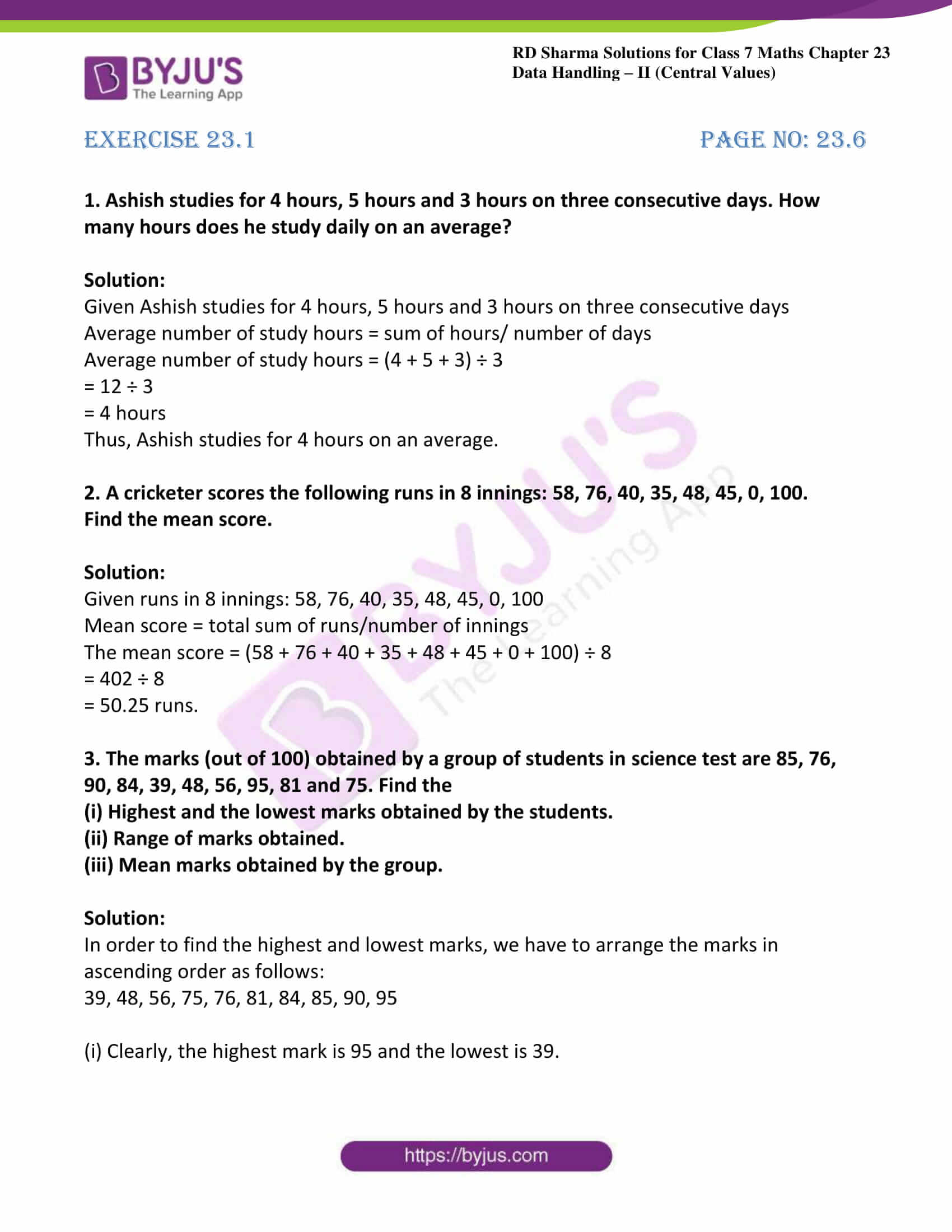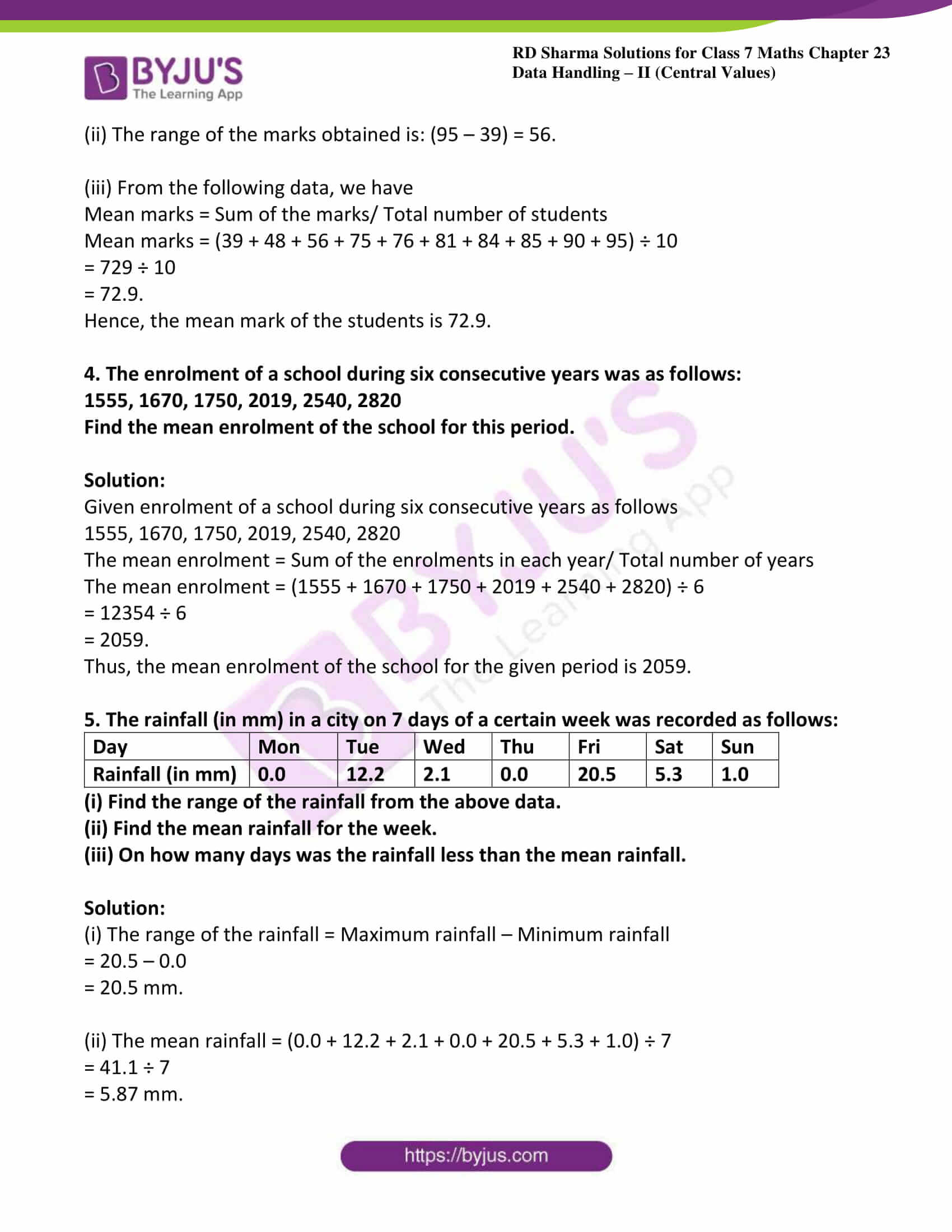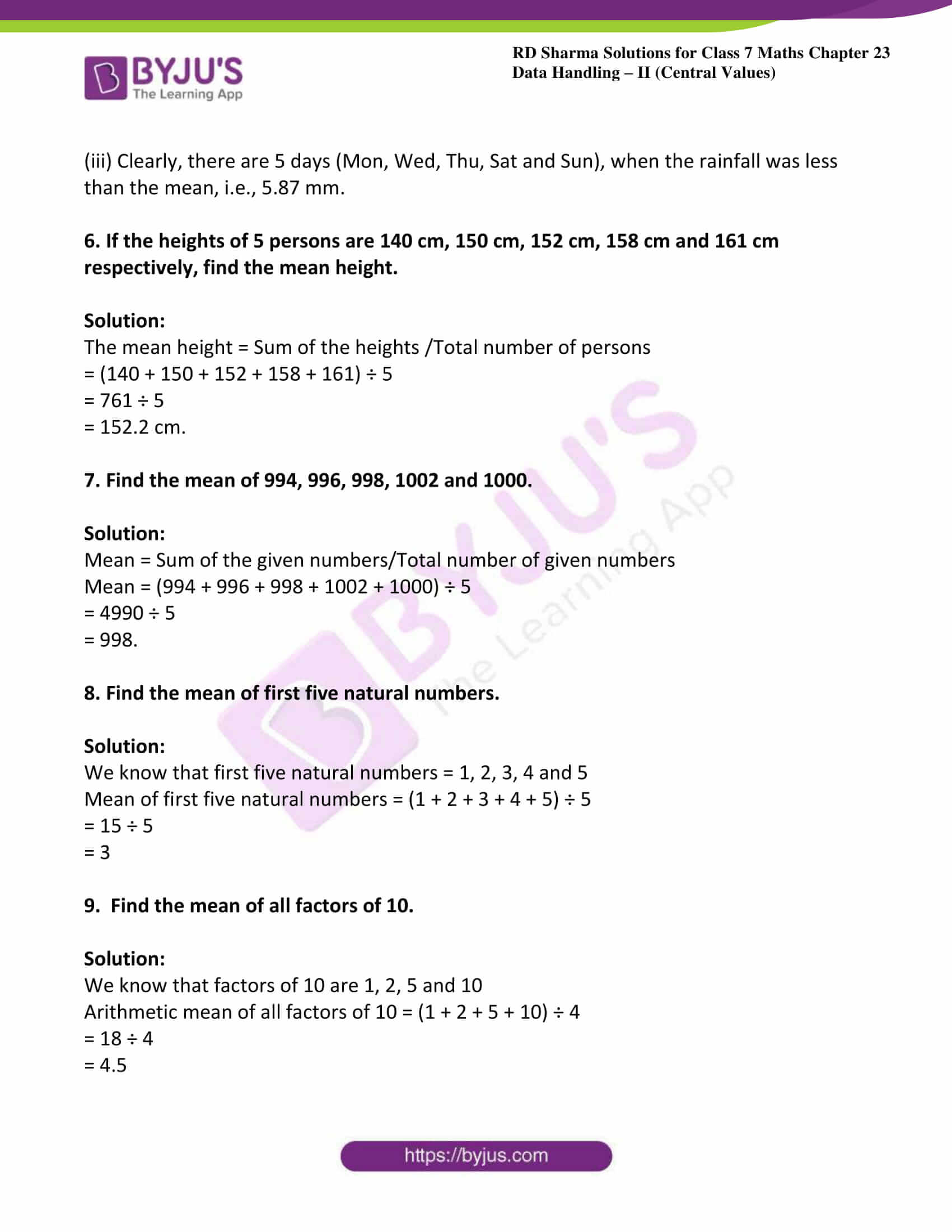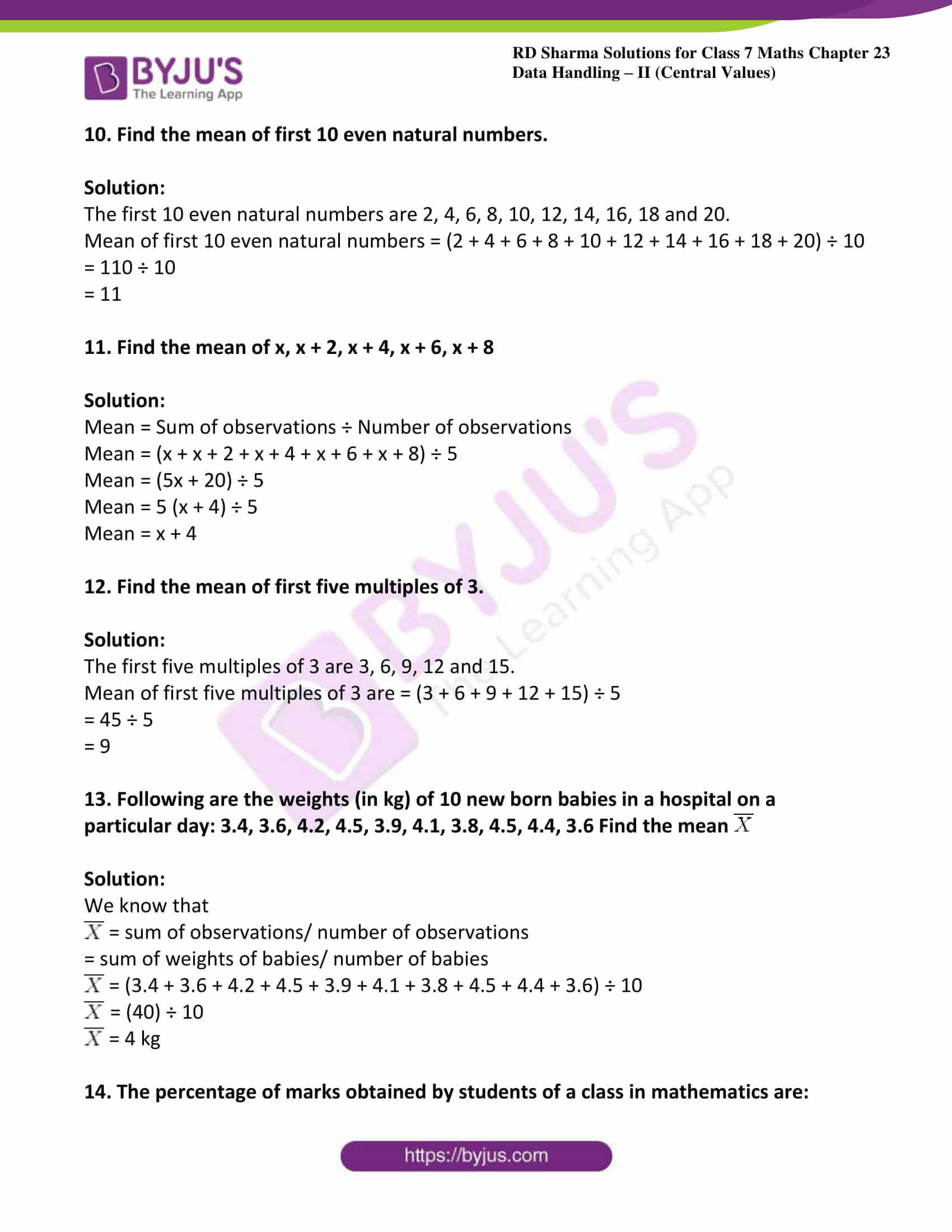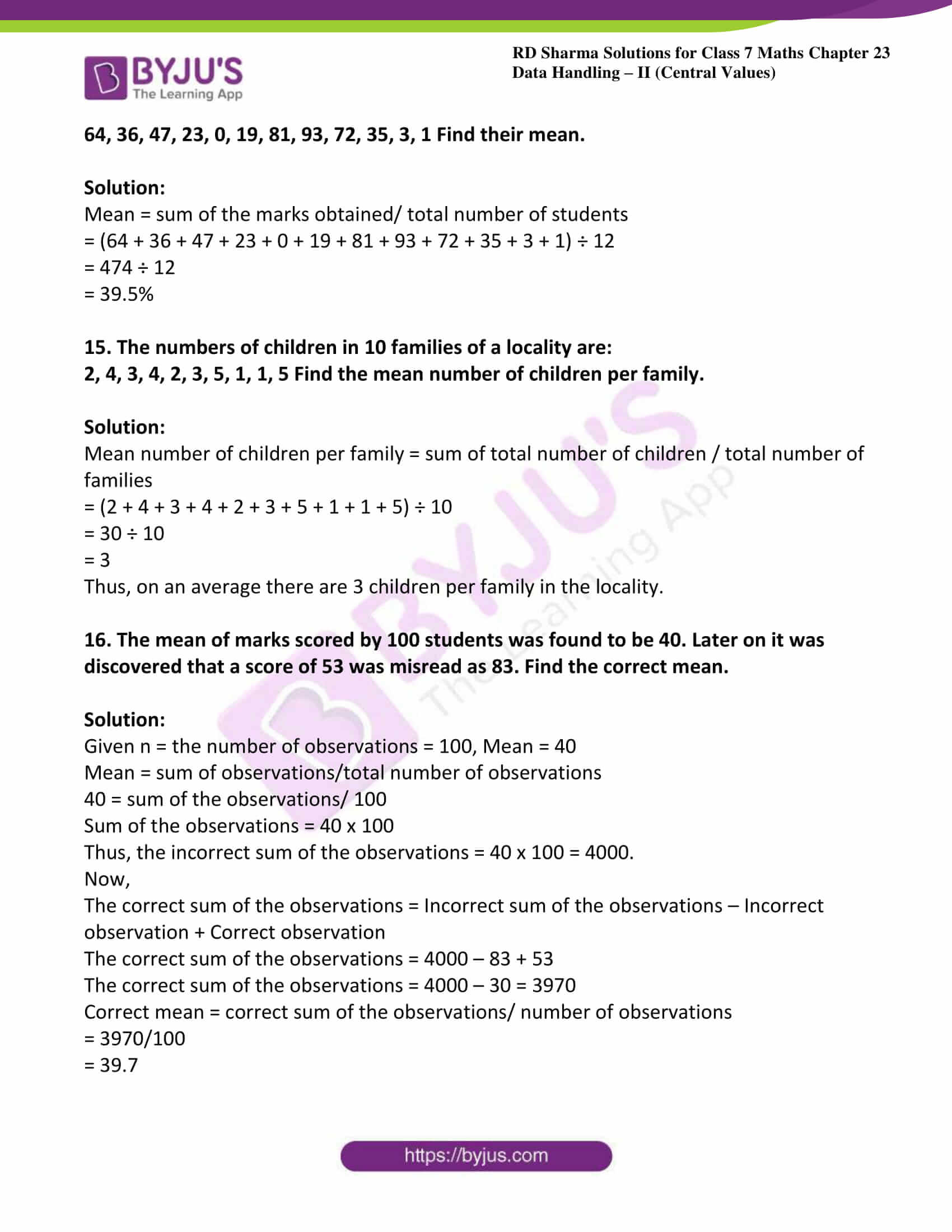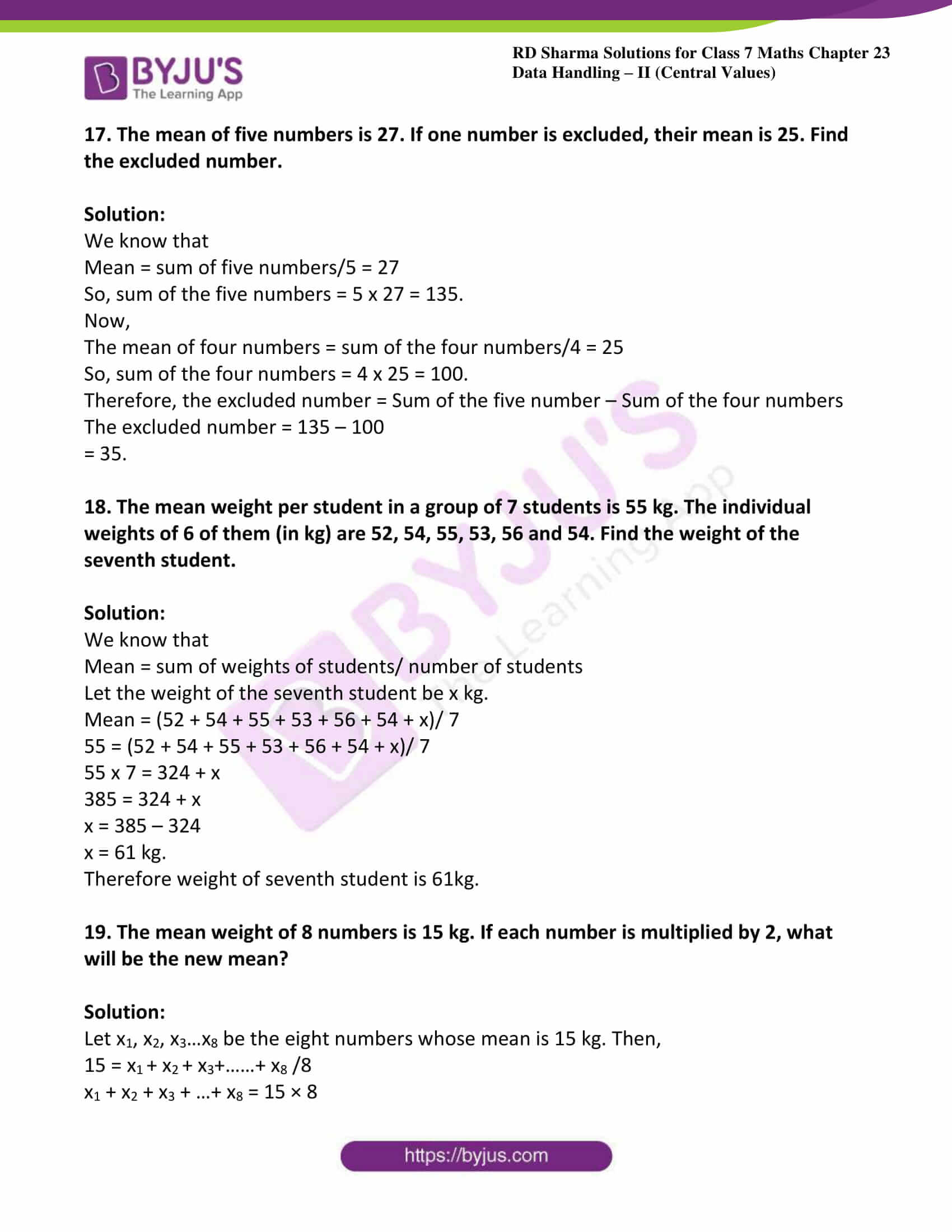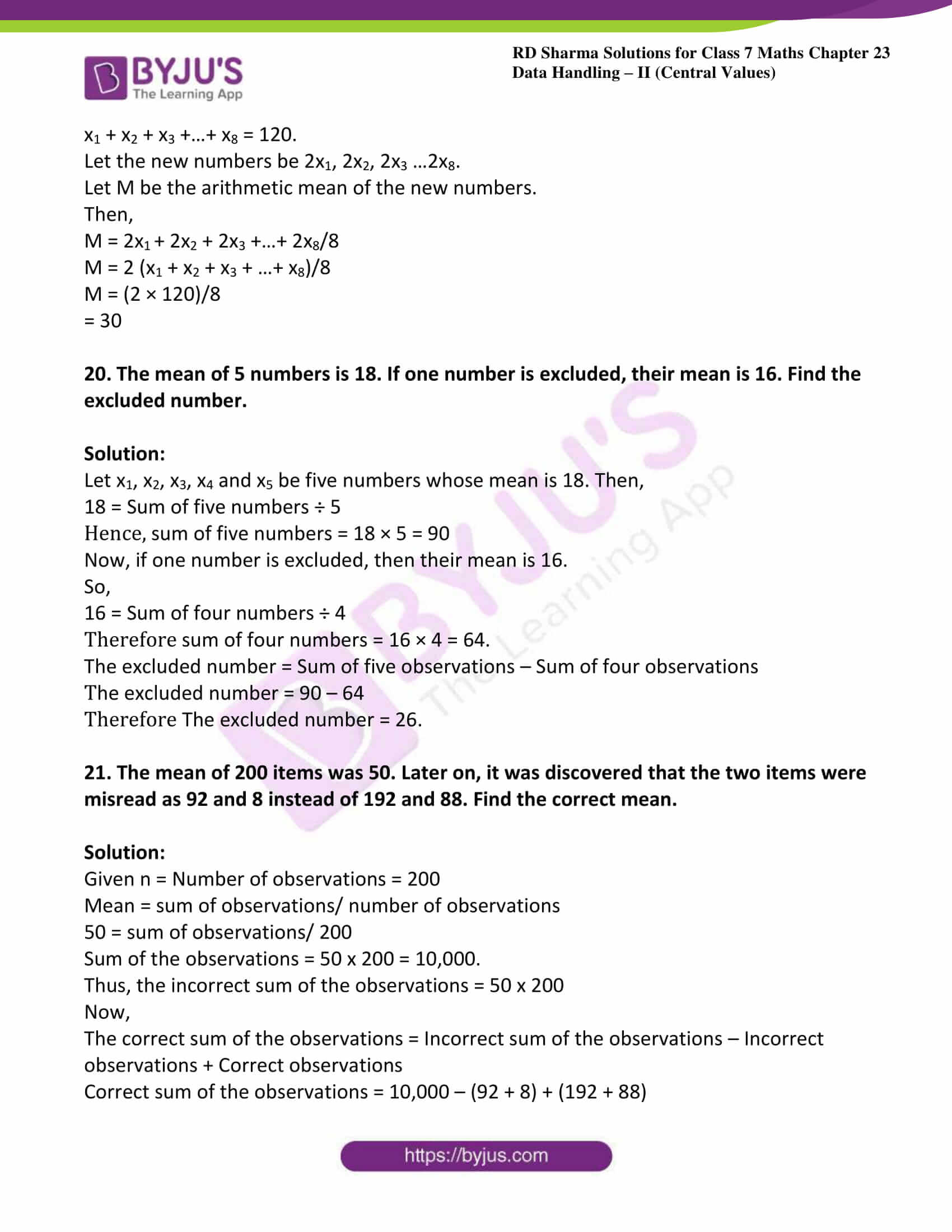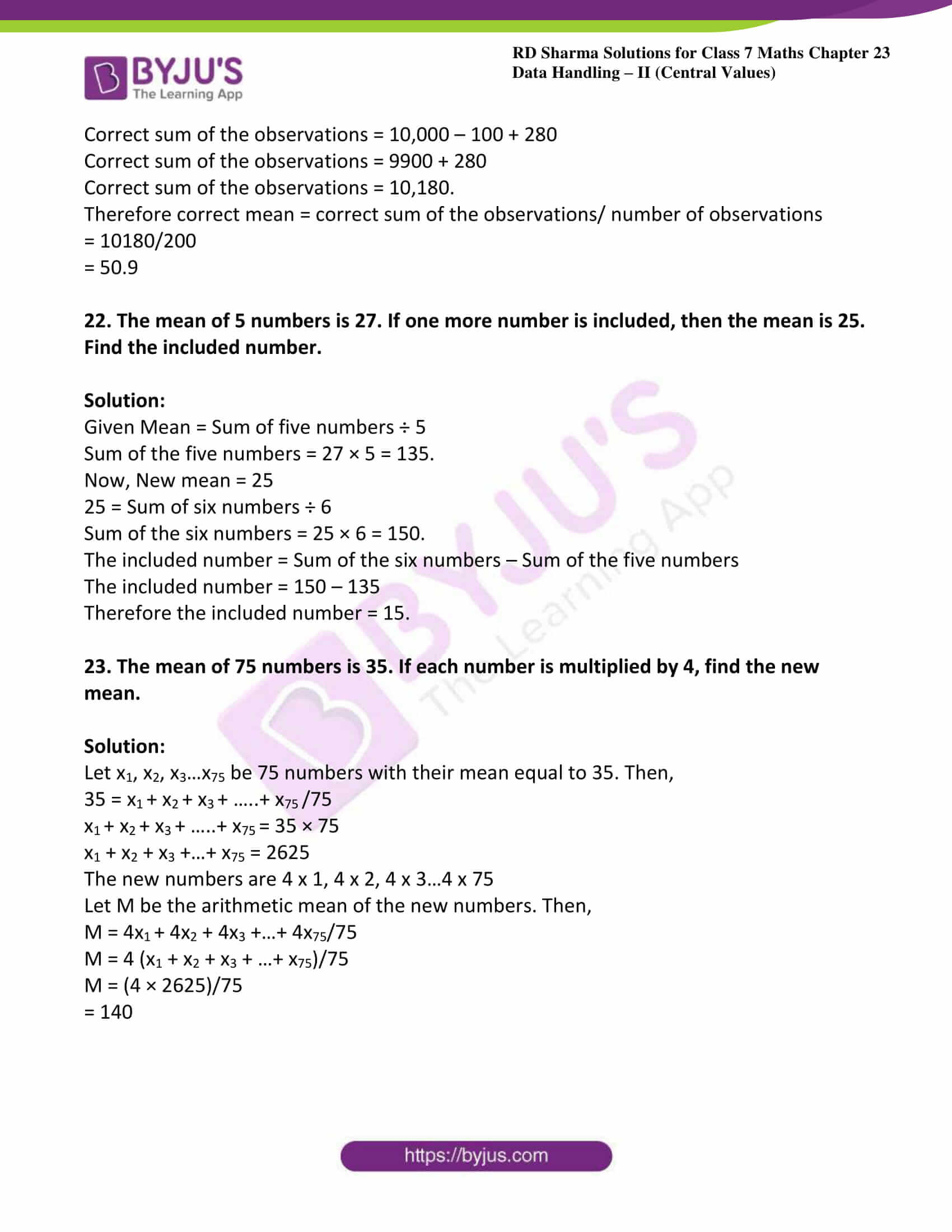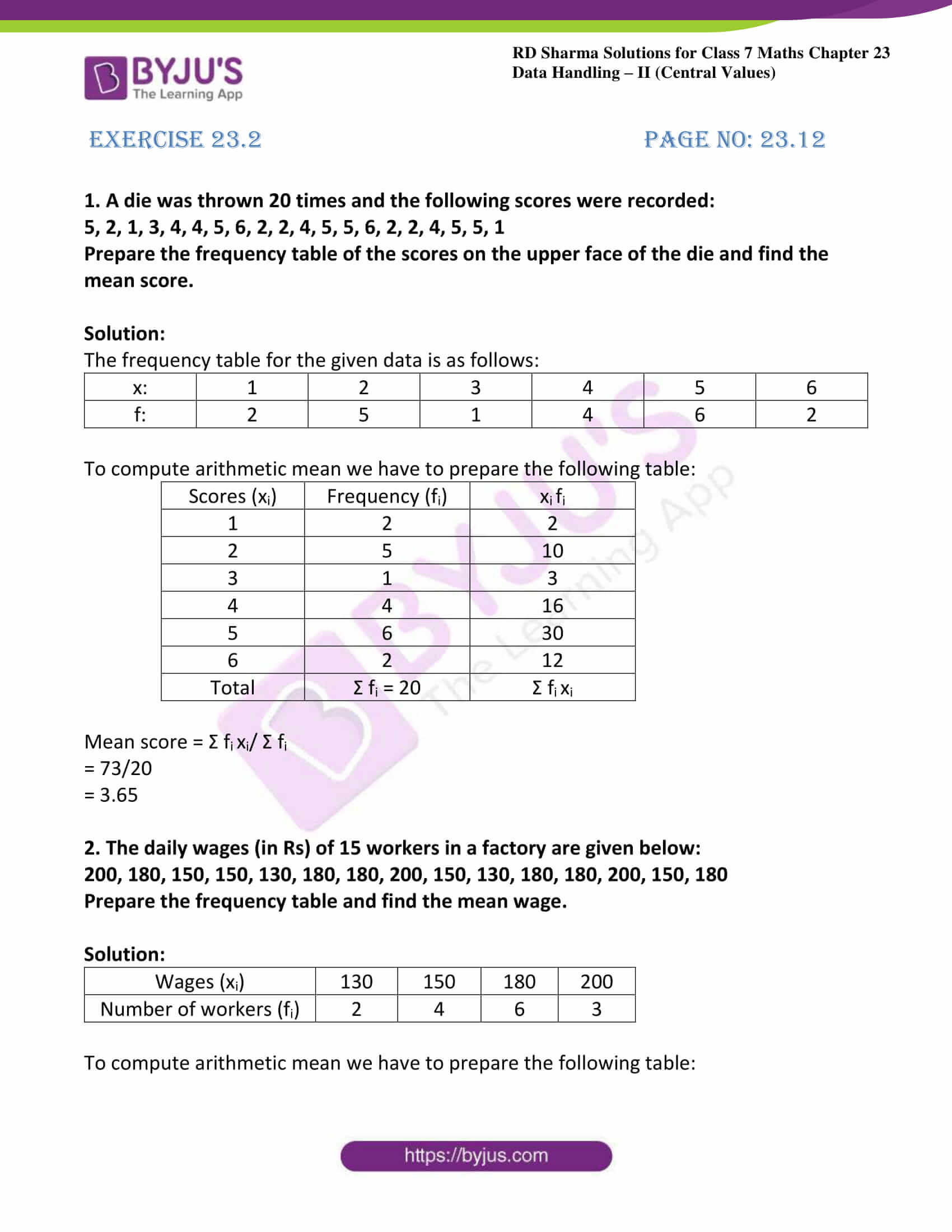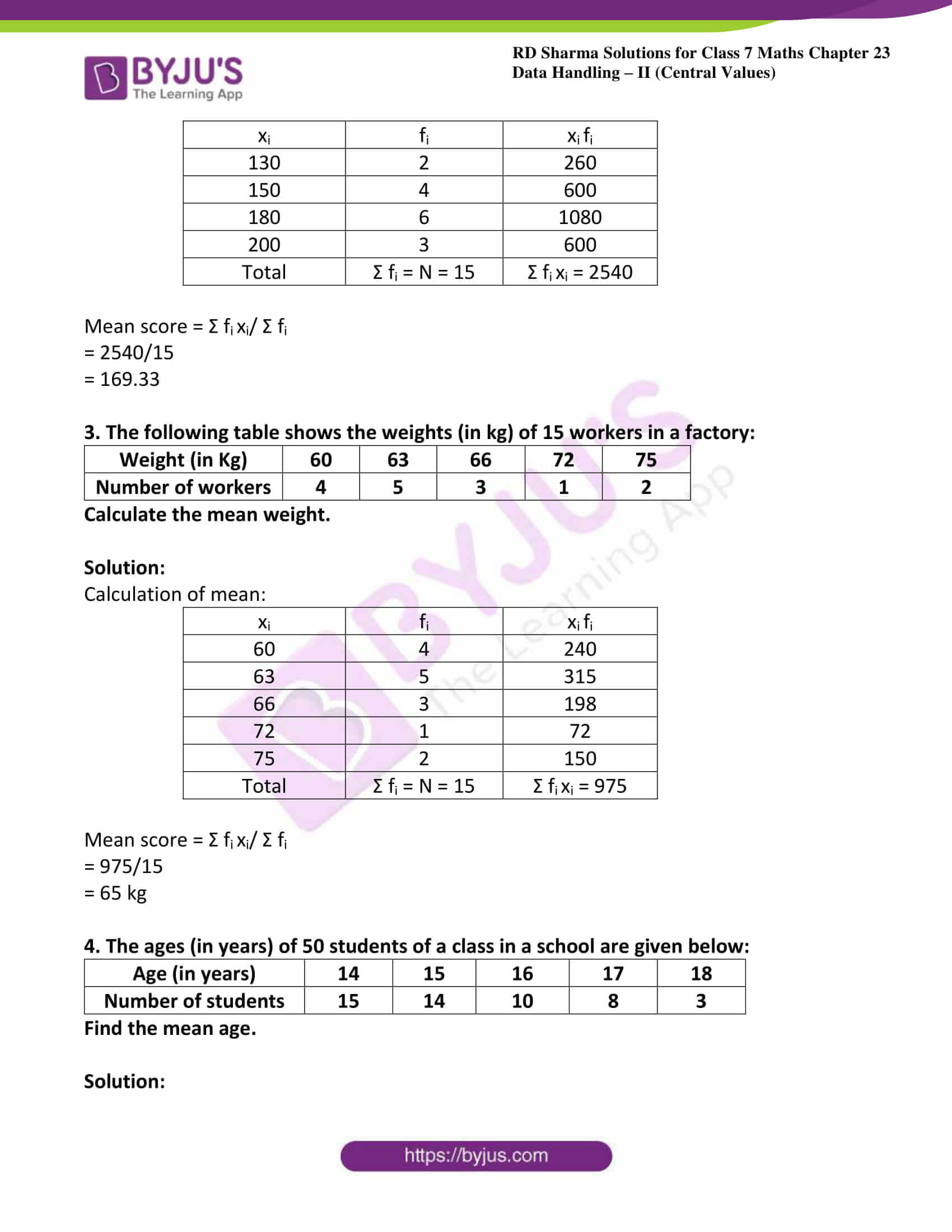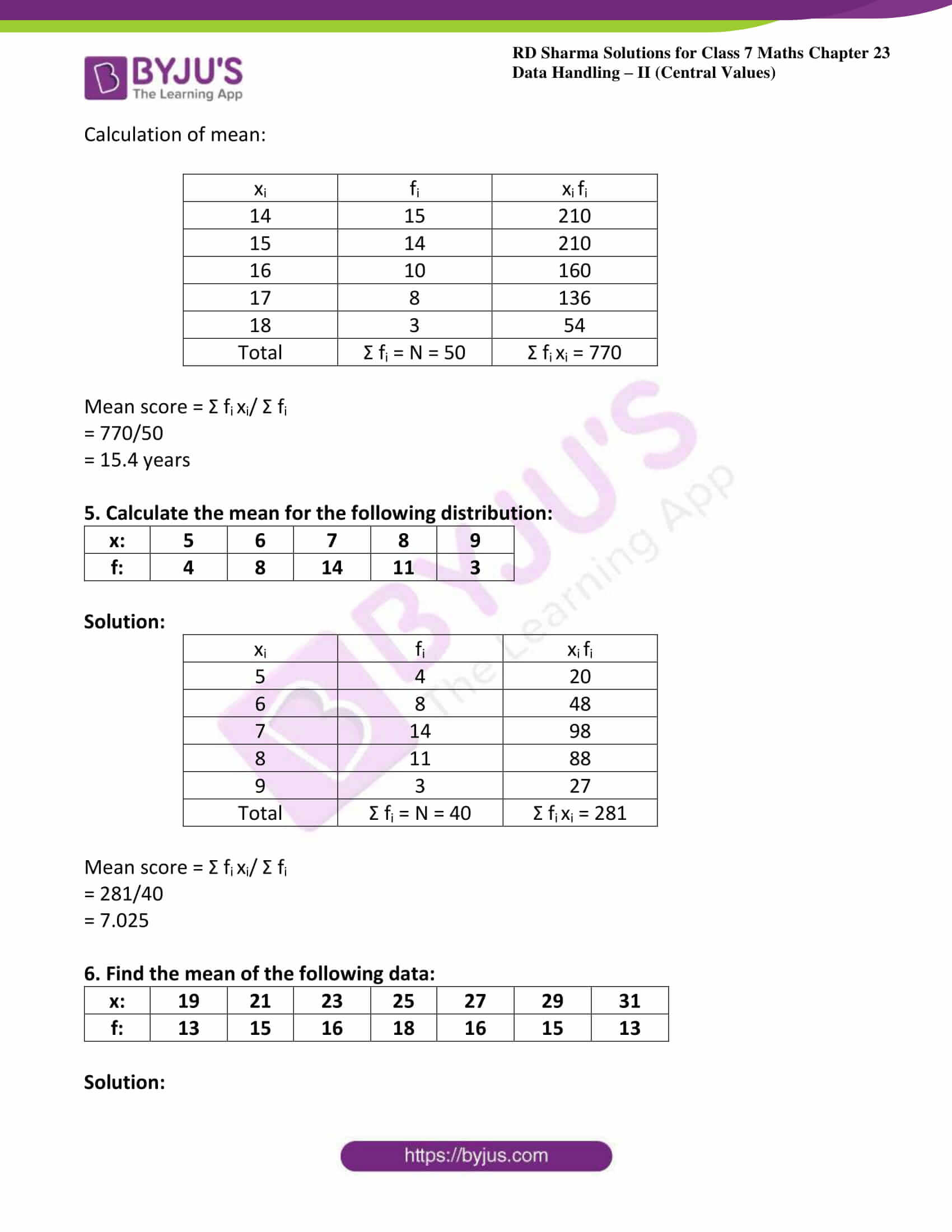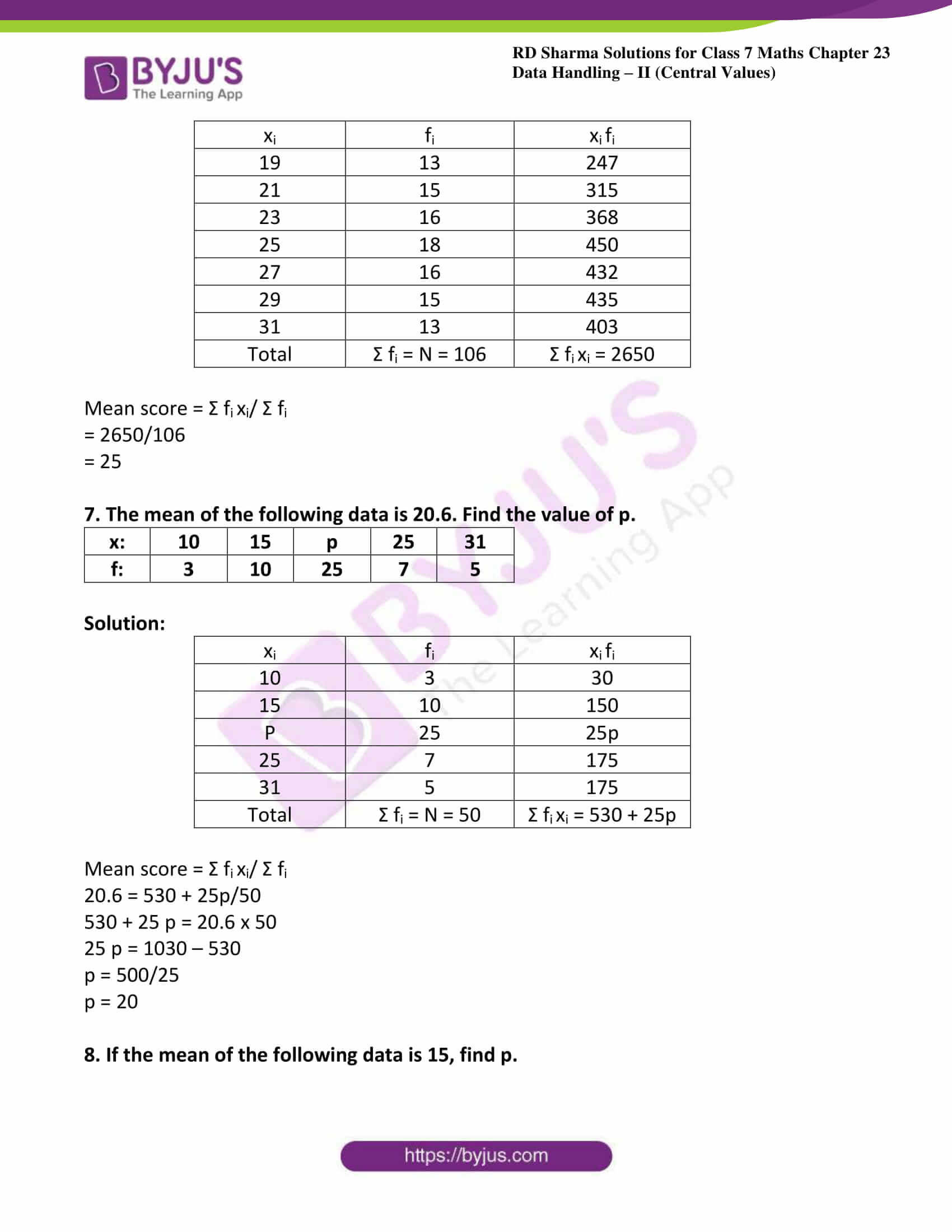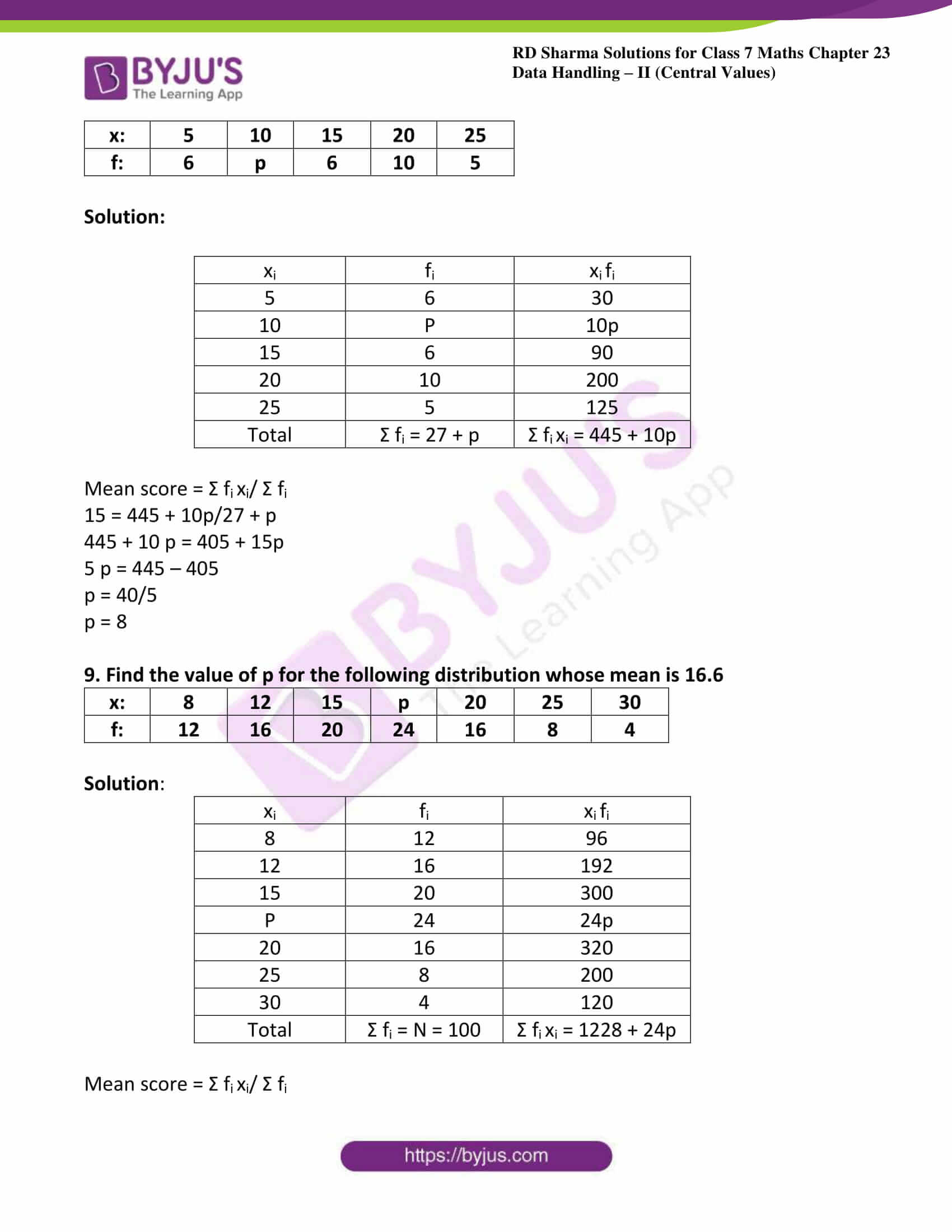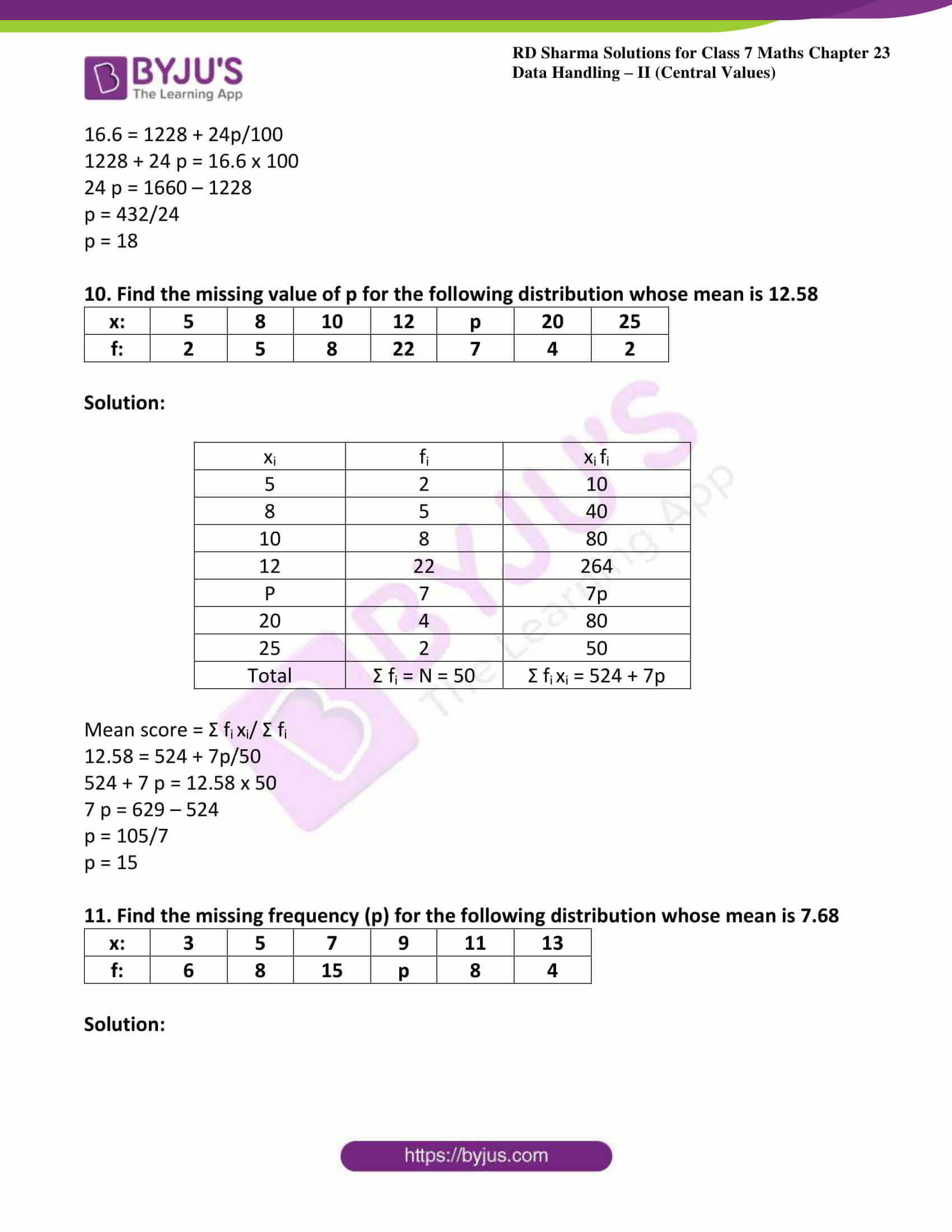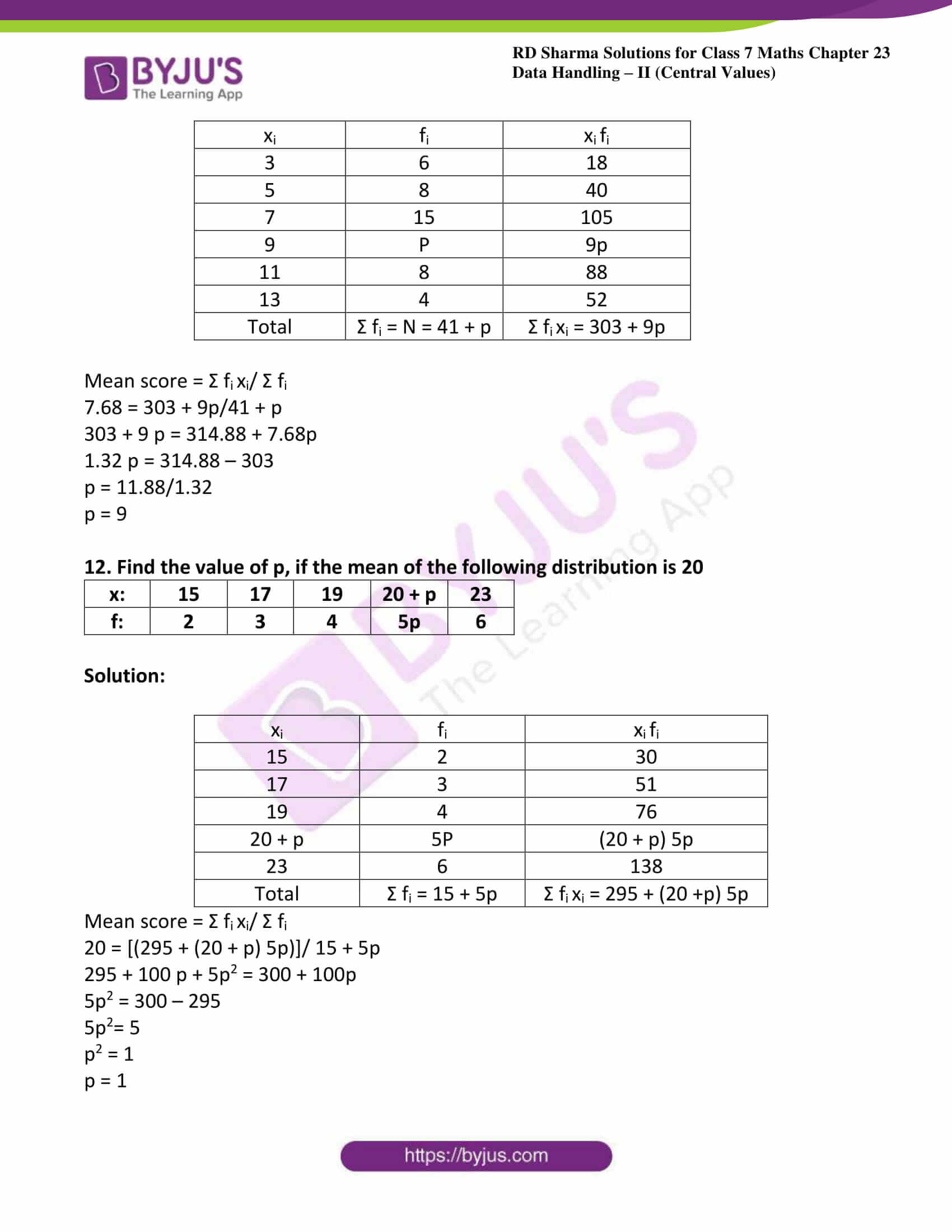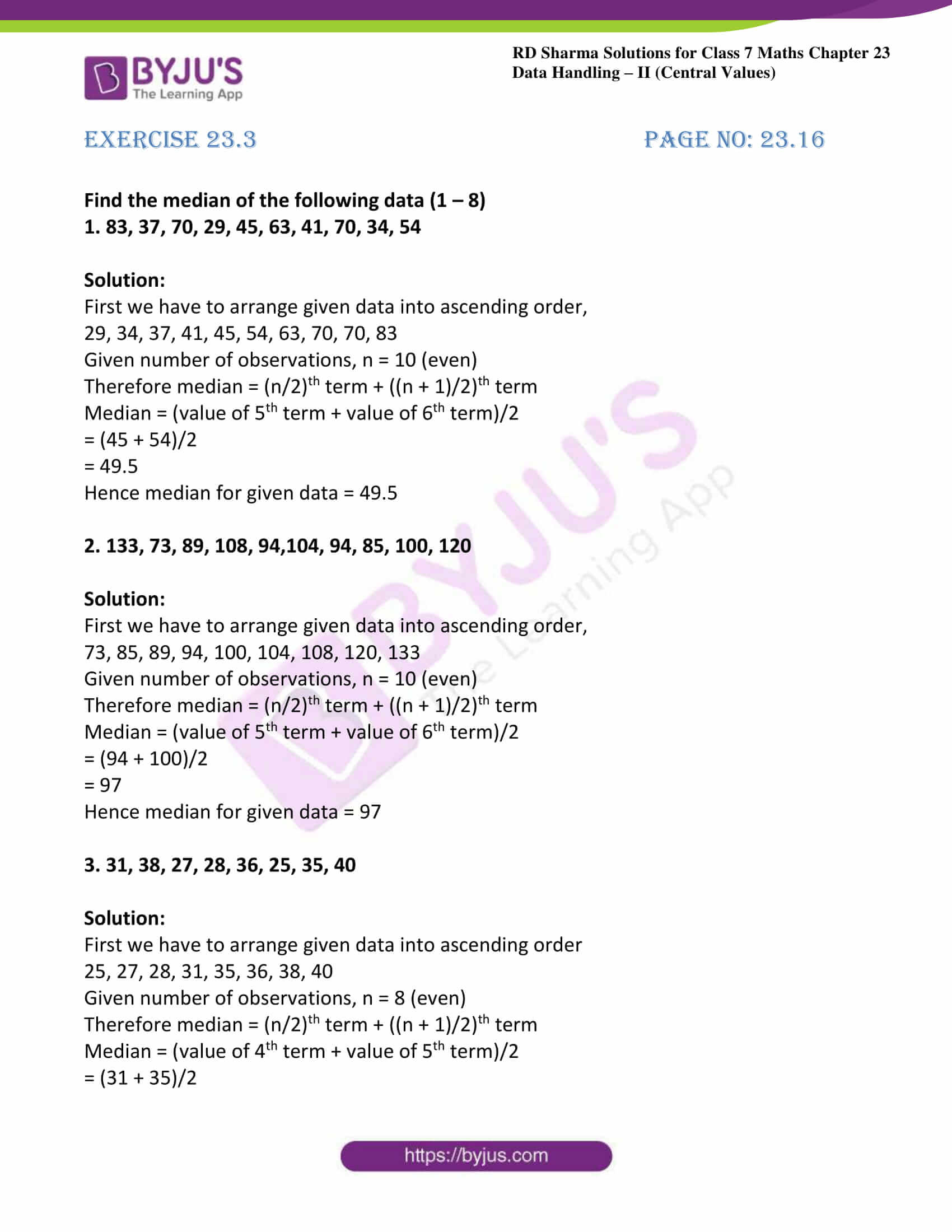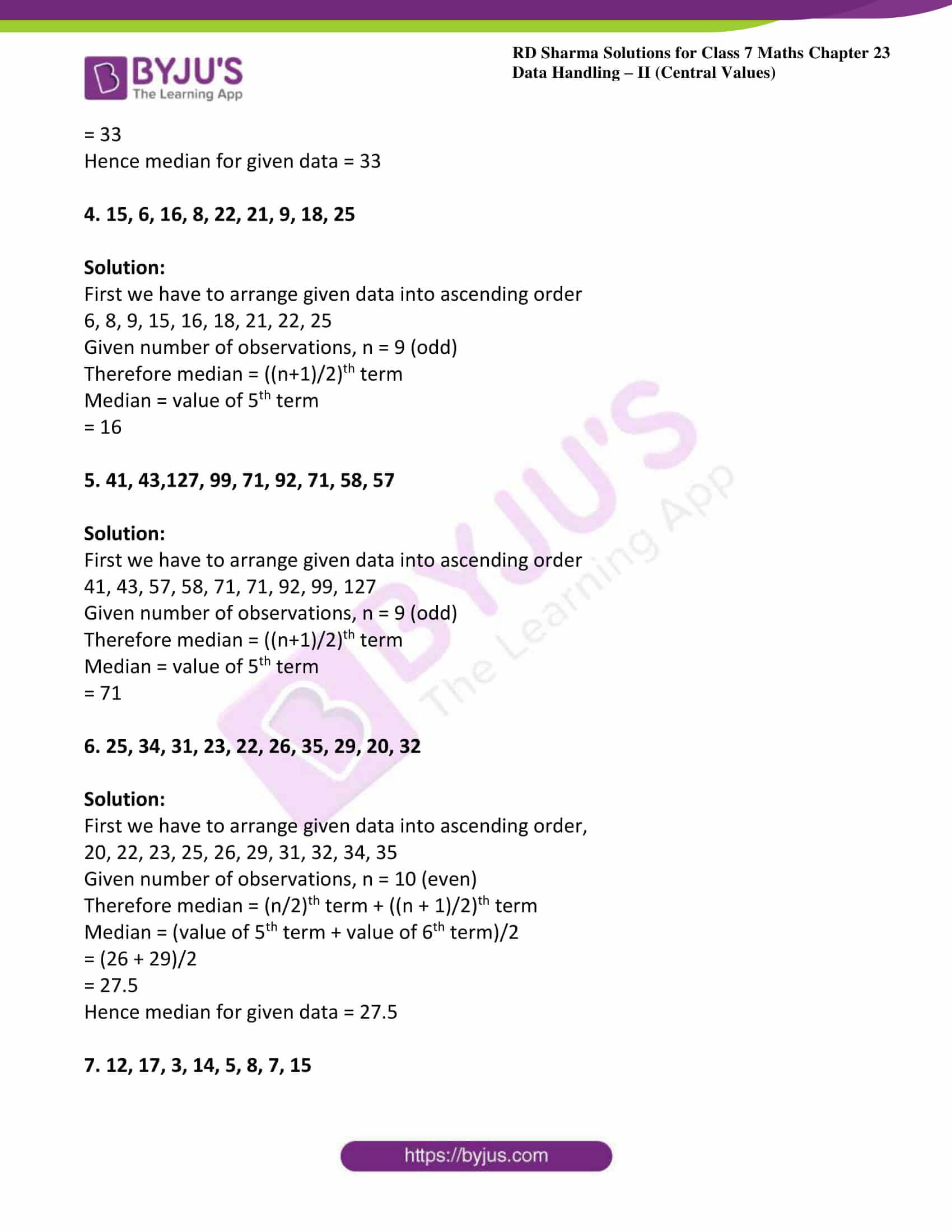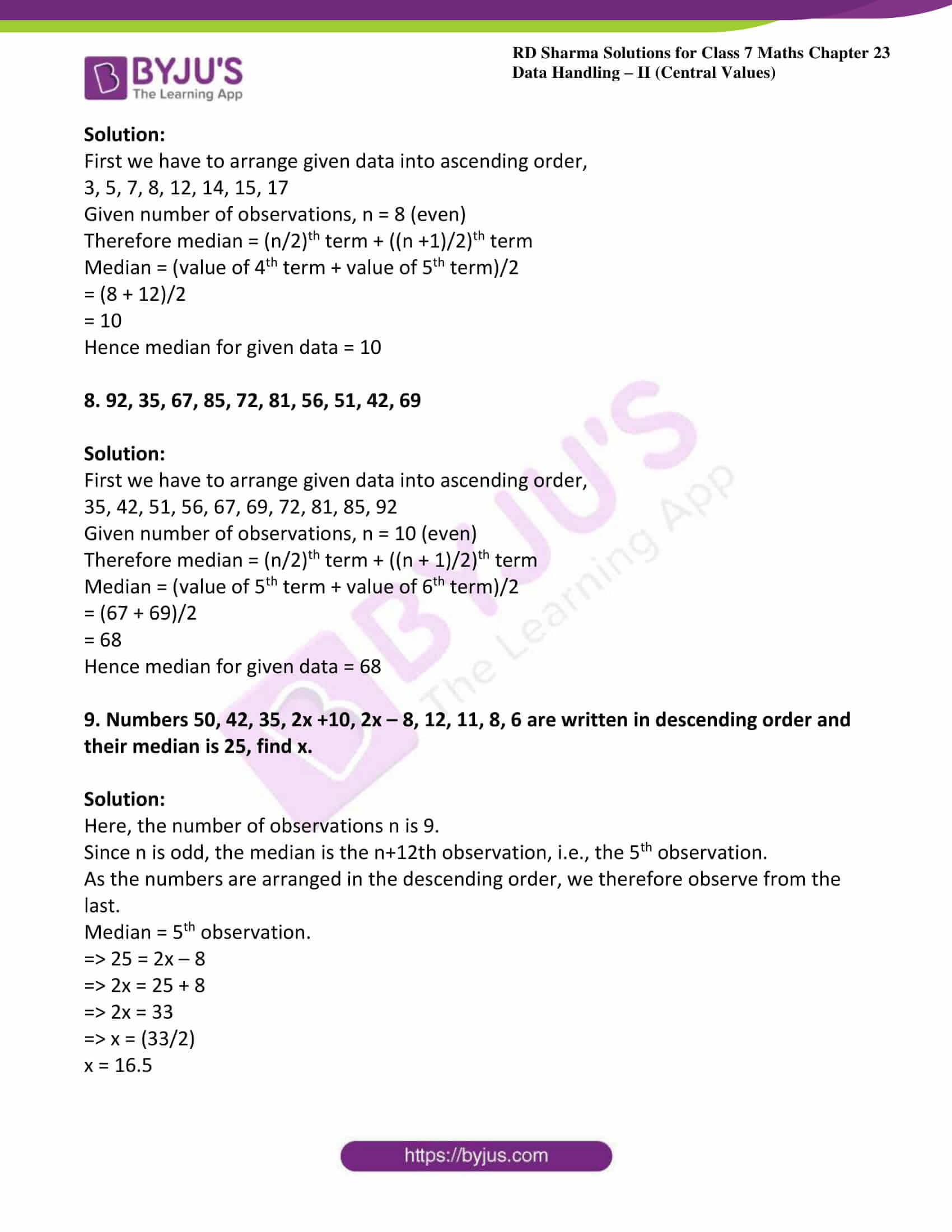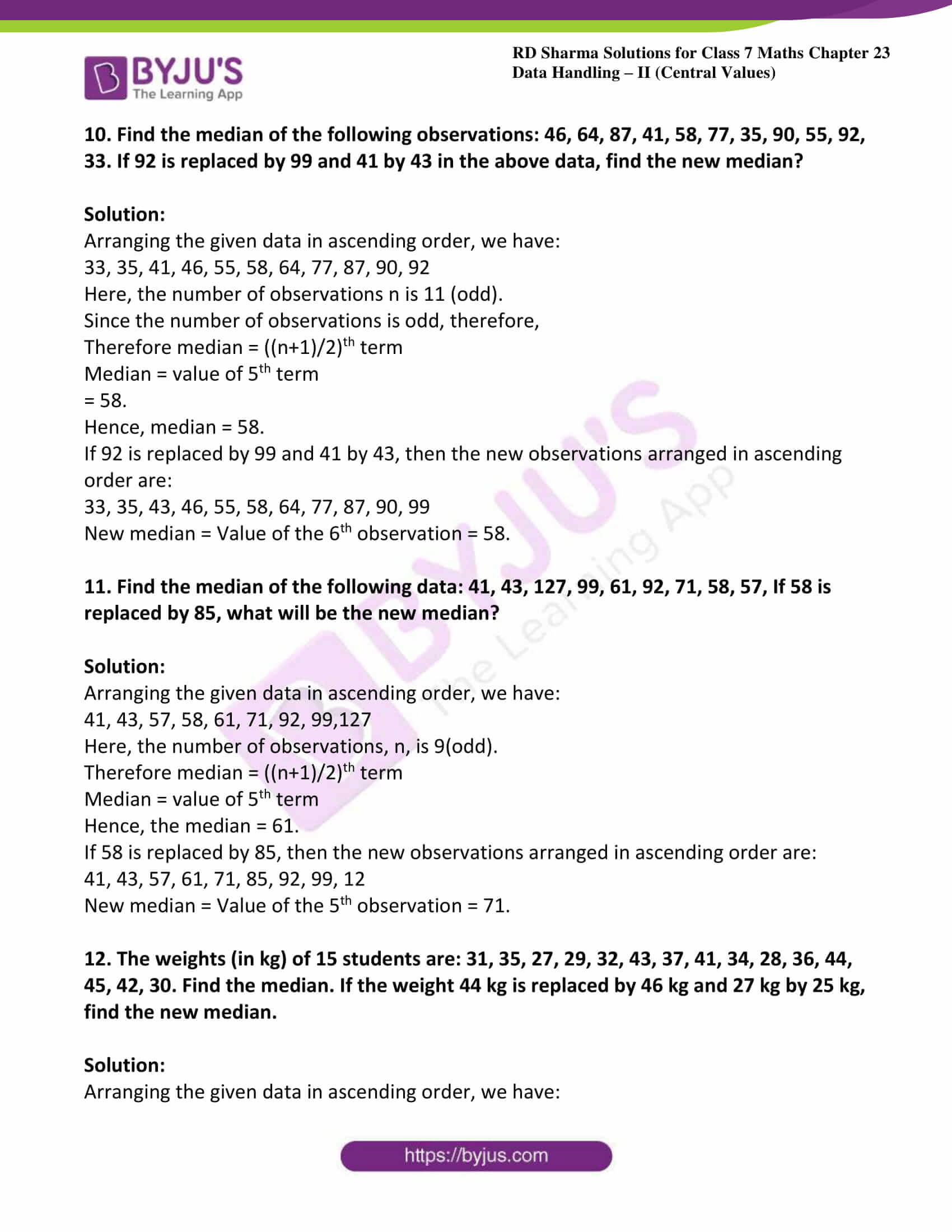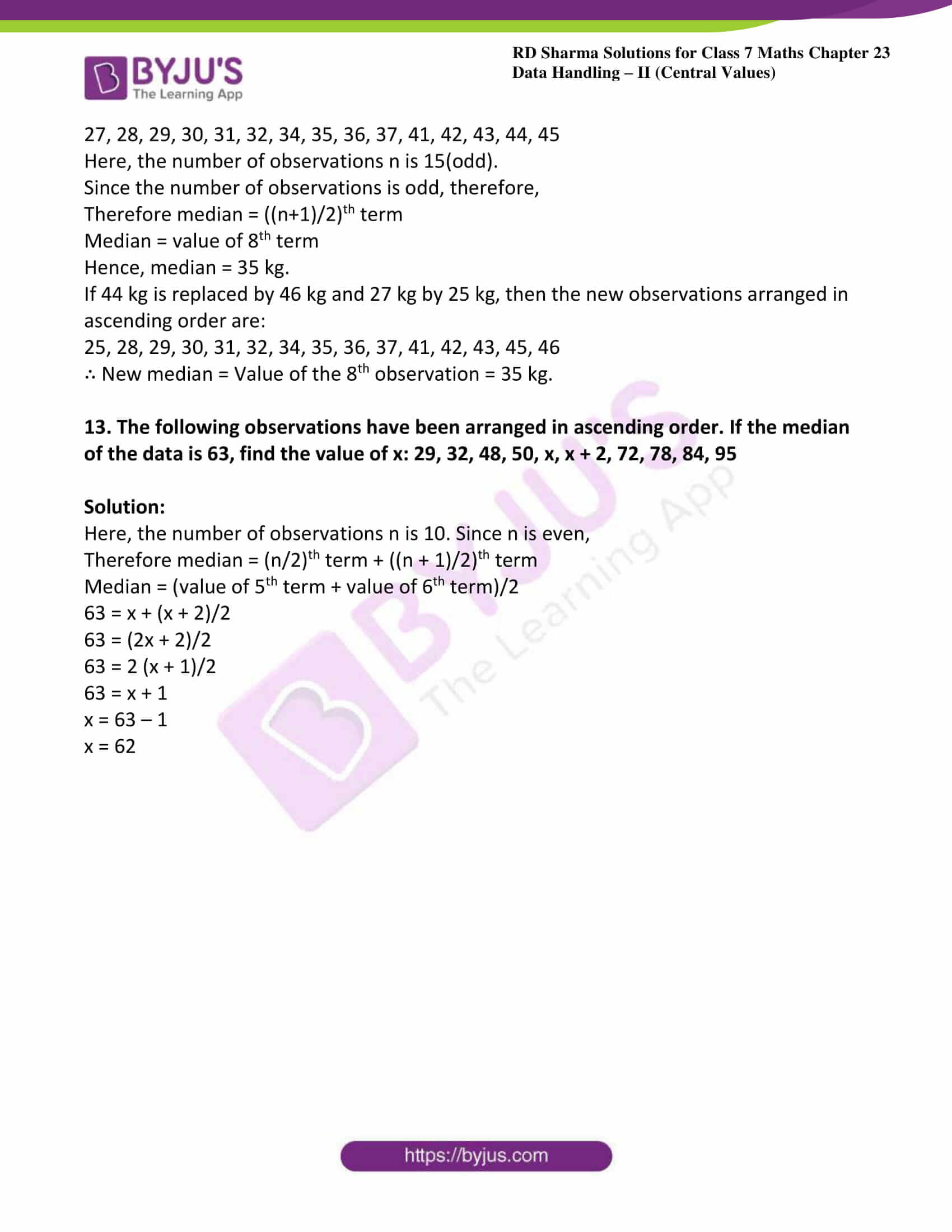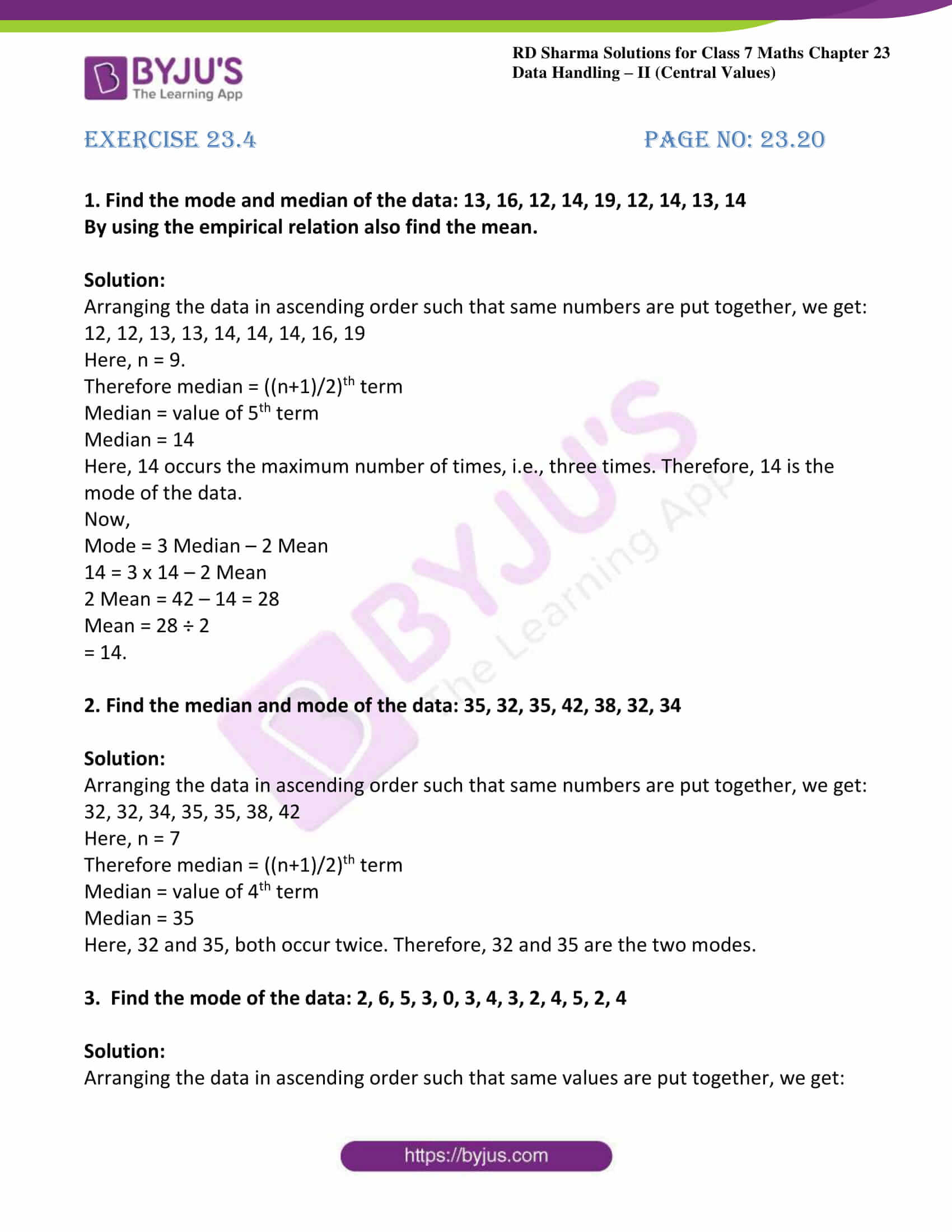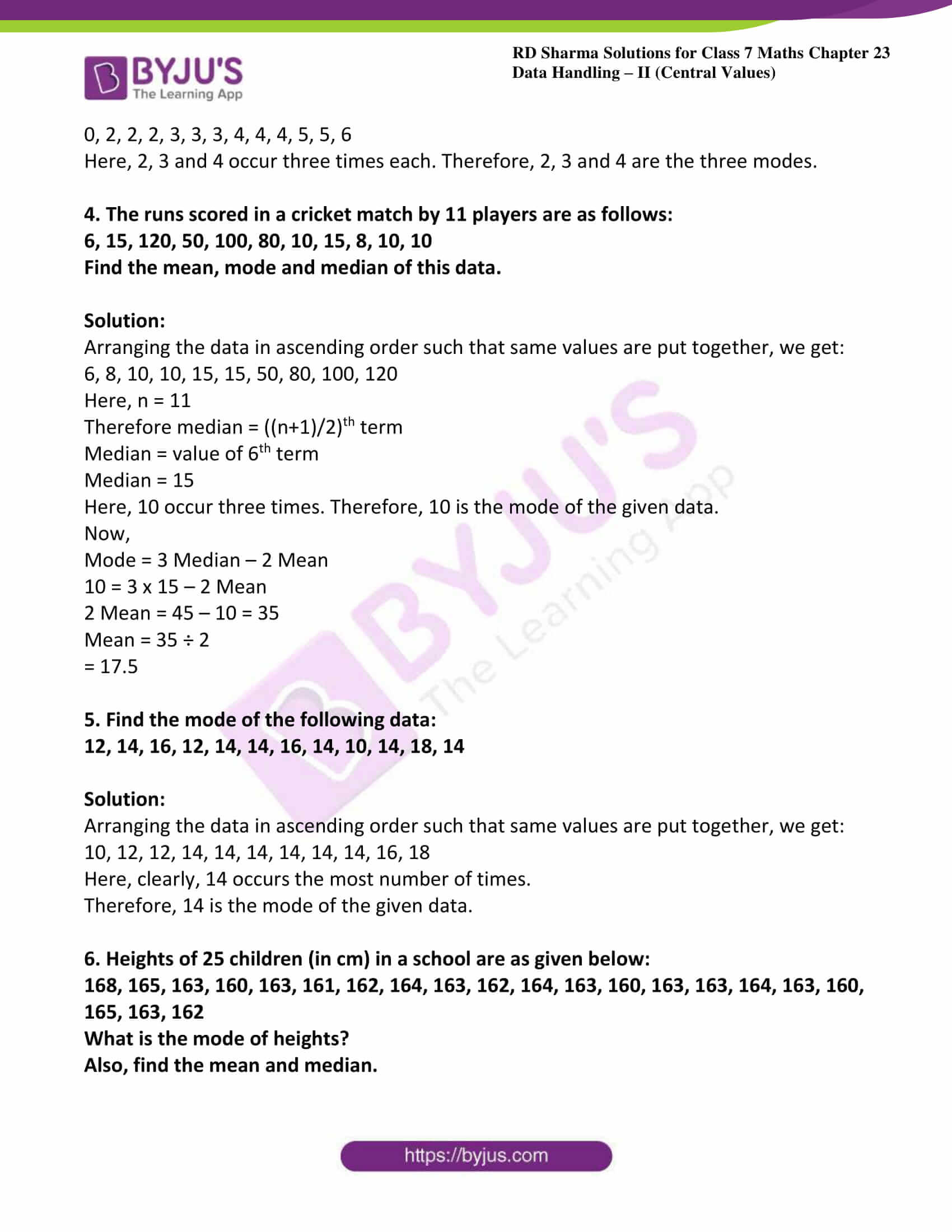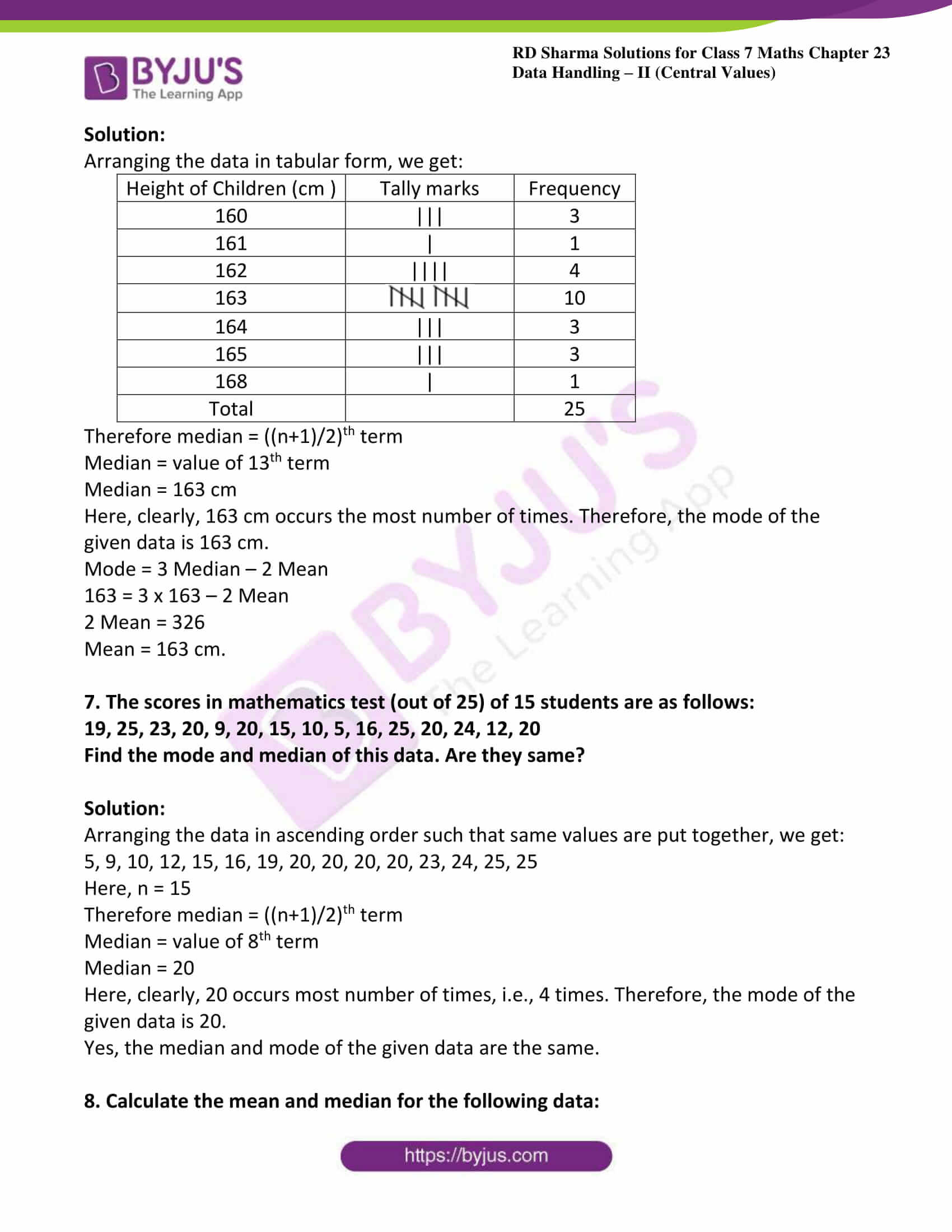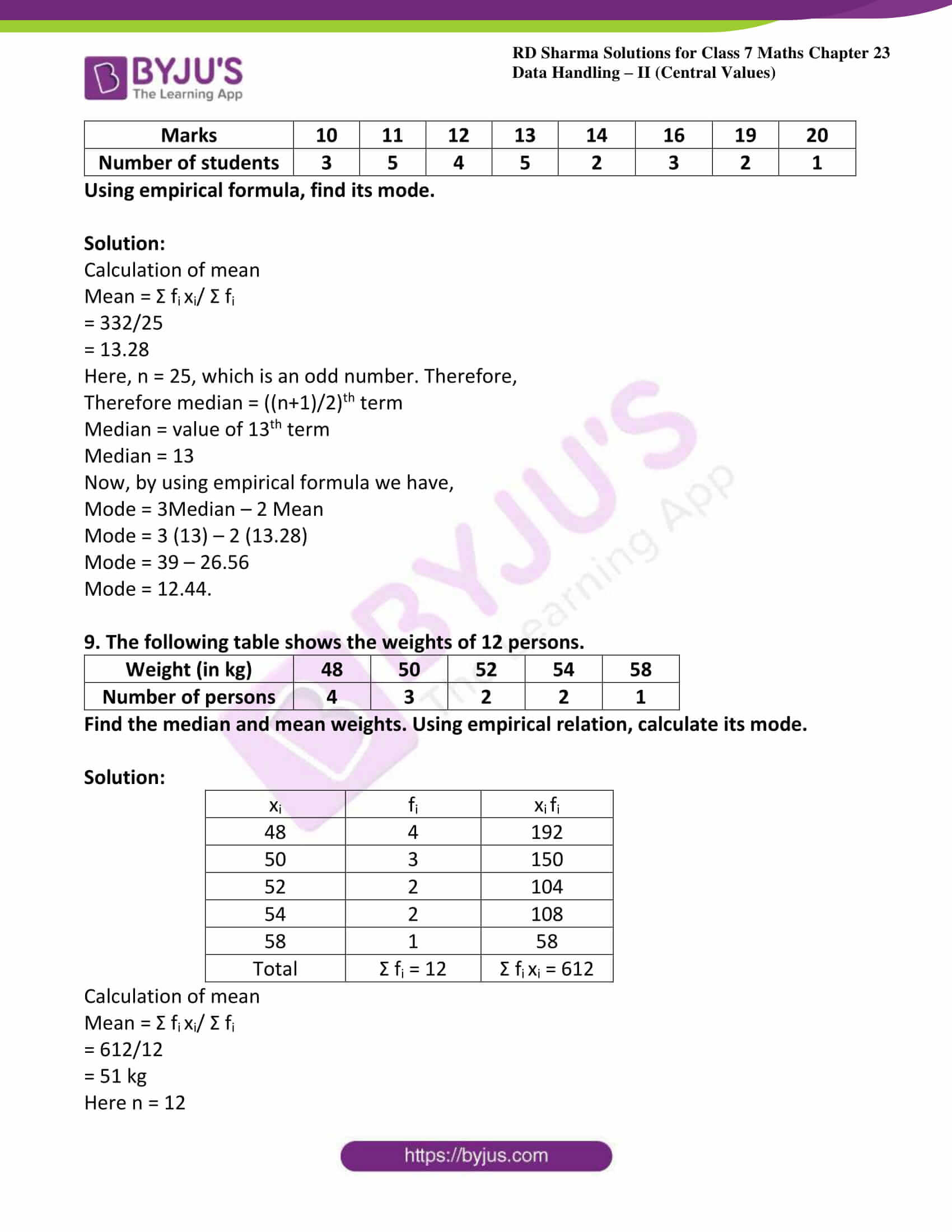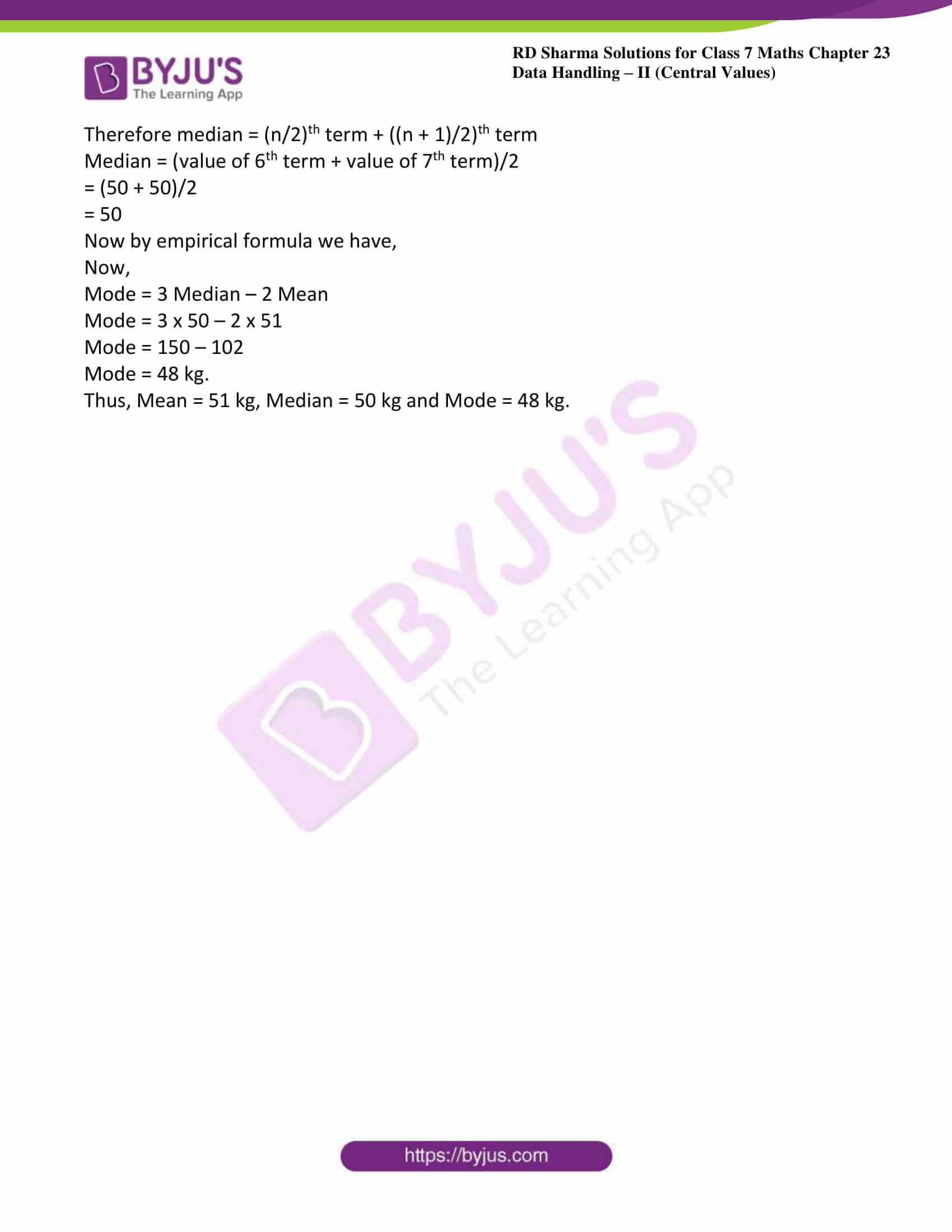### Access answers to Maths RD Sharma Solutions For Class 7 Chapter 23 – Data Handling – II (Central Values)

Exercise 23.1 Page No: 23.6

1. Ashish studies for 4 hours, 5 hours and 3 hours on three consecutive days. How many hours does he study daily on an average?

Solution:

Given Ashish studies for 4 hours, 5 hours and 3 hours on three consecutive days

Average number of study hours = sum of hours/ number of days

Average number of study hours = (4 + 5 + 3) ÷ 3

= 12 ÷ 3

= 4 hours

Thus, Ashish studies for 4 hours on an average.

2. A cricketer scores the following runs in 8 innings: 58, 76, 40, 35, 48, 45, 0, 100.

Find the mean score.

Solution:

Given runs in 8 innings: 58, 76, 40, 35, 48, 45, 0, 100

Mean score = total sum of runs/number of innings

The mean score = (58 + 76 + 40 + 35 + 48 + 45 + 0 + 100) ÷ 8

= 402 ÷ 8

= 50.25 runs.

3. The marks (out of 100) obtained by a group of students in science test are 85, 76, 90, 84, 39, 48, 56, 95, 81 and 75. Find the

(i) Highest and the lowest marks obtained by the students.

(ii) Range of marks obtained.

(iii) Mean marks obtained by the group.

Solution:

In order to find the highest and lowest marks, we have to arrange the marks in ascending order as follows:

39, 48, 56, 75, 76, 81, 84, 85, 90, 95

(i) Clearly, the highest mark is 95 and the lowest is 39.

(ii) The range of the marks obtained is: (95 – 39) = 56.

(iii) From the following data, we have

Mean marks = Sum of the marks/ Total number of students

Mean marks = (39 + 48 + 56 + 75 + 76 + 81 + 84 + 85 + 90 + 95) ÷ 10

= 729 ÷ 10

= 72.9.

Hence, the mean mark of the students is 72.9.

4. The enrolment of a school during six consecutive years was as follows:

1555, 1670, 1750, 2019, 2540, 2820

Find the mean enrolment of the school for this period.

Solution:

Given enrolment of a school during six consecutive years as follows

1555, 1670, 1750, 2019, 2540, 2820

The mean enrolment = Sum of the enrolments in each year/ Total number of years

The mean enrolment = (1555 + 1670 + 1750 + 2019 + 2540 + 2820) ÷ 6

= 12354 ÷ 6

= 2059.

Thus, the mean enrolment of the school for the given period is 2059.

5. The rainfall (in mm) in a city on 7 days of a certain week was recorded as follows:

 Day Mon Tue Wed Thu Fri Sat Sun Rainfall (in mm) 0.0 12.2 2.1 0.0 20.5 5.3 1.0

(i) Find the range of the rainfall from the above data.

(ii) Find the mean rainfall for the week.

(iii) On how many days was the rainfall less than the mean rainfall.

Solution:

(i) The range of the rainfall = Maximum rainfall – Minimum rainfall

= 20.5 – 0.0

= 20.5 mm.

(ii) The mean rainfall = (0.0 + 12.2 + 2.1 + 0.0 + 20.5 + 5.3 + 1.0) ÷ 7

= 41.1 ÷ 7

= 5.87 mm.

(iii) Clearly, there are 5 days (Mon, Wed, Thu, Sat and Sun), when the rainfall was less than the mean, i.e., 5.87 mm.

6. If the heights of 5 persons are 140 cm, 150 cm, 152 cm, 158 cm and 161 cm respectively, find the mean height.

Solution:

The mean height = Sum of the heights /Total number of persons

= (140 + 150 + 152 + 158 + 161) ÷ 5

= 761 ÷ 5

= 152.2 cm.

7. Find the mean of 994, 996, 998, 1002 and 1000.

Solution:

Mean = Sum of the given numbers/Total number of given numbers

Mean = (994 + 996 + 998 + 1002 + 1000) ÷ 5

= 4990 ÷ 5

= 998.

8. Find the mean of first five natural numbers.

Solution:

We know that first five natural numbers = 1, 2, 3, 4 and 5

Mean of first five natural numbers = (1 + 2 + 3 + 4 + 5) ÷ 5

= 15 ÷ 5

= 3

9. Find the mean of all factors of 10.

Solution:

We know that factors of 10 are 1, 2, 5 and 10

Arithmetic mean of all factors of 10 = (1 + 2 + 5 + 10) ÷ 4

= 18 ÷ 4

= 4.5

10. Find the mean of first 10 even natural numbers.

Solution:

The first 10 even natural numbers are 2, 4, 6, 8, 10, 12, 14, 16, 18 and 20.

Mean of first 10 even natural numbers = (2 + 4 + 6 + 8 + 10 + 12 + 14 + 16 + 18 + 20) ÷ 10

= 110 ÷ 10

= 11

11. Find the mean of x, x + 2, x + 4, x + 6, x + 8

Solution:

Mean = Sum of observations ÷ Number of observations

Mean = (x + x + 2 + x + 4 + x + 6 + x + 8) ÷ 5

Mean = (5x + 20) ÷ 5

Mean = 5 (x + 4) ÷ 5

Mean = x + 4

12. Find the mean of first five multiples of 3.

Solution:

The first five multiples of 3 are 3, 6, 9, 12 and 15.

Mean of first five multiples of 3 are = (3 + 6 + 9 + 12 + 15) ÷ 5

= 45 ÷ 5

= 9

13. Following are the weights (in kg) of 10 new born babies in a hospital on a particular day: 3.4, 3.6, 4.2, 4.5, 3.9, 4.1, 3.8, 4.5, 4.4, 3.6 Find the mean ${\overline{X}}$

Solution:

We know that

${\overline{X}}$ = sum of observations/ number of observations

${\overline{X}}$ = sum of weights of babies/ number of babies

${\overline{X}}$ = (3.4 + 3.6 + 4.2 + 4.5 + 3.9 + 4.1 + 3.8 + 4.5 + 4.4 + 3.6) ÷ 10

${\overline{X}}$ = (40) ÷ 10

${\overline{X}}$ = 4 kg

14. The percentage of marks obtained by students of a class in mathematics are:

64, 36, 47, 23, 0, 19, 81, 93, 72, 35, 3, 1 Find their mean.

Solution:

Mean = sum of the marks obtained/ total number of students

= (64 + 36 + 47 + 23 + 0 + 19 + 81 + 93 + 72 + 35 + 3 + 1) ÷ 12

= 474 ÷ 12

= 39.5%

15. The numbers of children in 10 families of a locality are:

2, 4, 3, 4, 2, 3, 5, 1, 1, 5 Find the mean number of children per family.

Solution:

Mean number of children per family = sum of total number of children / total number of families

= (2 + 4 + 3 + 4 + 2 + 3 + 5 + 1 + 1 + 5) ÷ 10

= 30 ÷ 10

= 3

Thus, on an average there are 3 children per family in the locality.

16. The mean of marks scored by 100 students was found to be 40. Later on it was discovered that a score of 53 was misread as 83. Find the correct mean.

Solution:

Given n = the number of observations = 100, Mean = 40

Mean = sum of observations/total number of observations

40 = sum of the observations/ 100

Sum of the observations = 40 x 100

Thus, the incorrect sum of the observations = 40 x 100 = 4000.

Now,

The correct sum of the observations = Incorrect sum of the observations – Incorrect observation + Correct observation

The correct sum of the observations = 4000 – 83 + 53

The correct sum of the observations = 4000 – 30 = 3970

Correct mean = correct sum of the observations/ number of observations

= 3970/100

= 39.7

17. The mean of five numbers is 27. If one number is excluded, their mean is 25. Find the excluded number.

Solution:

We know that

Mean = sum of five numbers/5 = 27

So, sum of the five numbers = 5 x 27 = 135.

Now,

The mean of four numbers = sum of the four numbers/4 = 25

So, sum of the four numbers = 4 x 25 = 100.

Therefore, the excluded number = Sum of the five number – Sum of the four numbers

The excluded number = 135 – 100

= 35.

18. The mean weight per student in a group of 7 students is 55 kg. The individual weights of 6 of them (in kg) are 52, 54, 55, 53, 56 and 54. Find the weight of the seventh student.

Solution:

We know that

Mean = sum of weights of students/ number of students

Let the weight of the seventh student be x kg.

Mean = (52 + 54 + 55 + 53 + 56 + 54 + x)/ 7

55 = (52 + 54 + 55 + 53 + 56 + 54 + x)/ 7

55 x 7 = 324 + x

385 = 324 + x

x = 385 – 324

x = 61 kg.

Therefore weight of seventh student is 61kg.

19. The mean weight of 8 numbers is 15 kg. If each number is multiplied by 2, what will be the new mean?

Solution:

Let x1, x2, x3…x8 be the eight numbers whose mean is 15 kg. Then,

15 = x1 + x2 + x3+……+ x8 /8

x1 + x2 + x3 + …+ x8 = 15 × 8

x1 + x2 + x3 +…+ x8 = 120.

Let the new numbers be 2x1, 2x2, 2x3 …2x8.

Let M be the arithmetic mean of the new numbers.

Then,

M = 2x1 + 2x2 + 2x3 +…+ 2x8/8

M = 2 (x1 + x2 + x3 + …+ x8)/8

M = (2 × 120)/8

= 30

20. The mean of 5 numbers is 18. If one number is excluded, their mean is 16. Find the excluded number.

Solution:

Let x1, x2, x3, x4 and x5 be five numbers whose mean is 18. Then,

18 = Sum of five numbers ÷ 5

Hence, sum of five numbers = 18 × 5 = 90

Now, if one number is excluded, then their mean is 16.

So,

16 = Sum of four numbers ÷ 4

Therefore sum of four numbers = 16 × 4 = 64.

The excluded number = Sum of five observations – Sum of four observations

The excluded number = 90 – 64

Therefore The excluded number = 26.

21. The mean of 200 items was 50. Later on, it was discovered that the two items were misread as 92 and 8 instead of 192 and 88. Find the correct mean.

Solution:

Given n = Number of observations = 200

Mean = sum of observations/ number of observations

50 = sum of observations/ 200

Sum of the observations = 50 x 200 = 10,000.

Thus, the incorrect sum of the observations = 50 x 200

Now,

The correct sum of the observations = Incorrect sum of the observations – Incorrect observations + Correct observations

Correct sum of the observations = 10,000 – (92 + 8) + (192 + 88)

Correct sum of the observations = 10,000 – 100 + 280

Correct sum of the observations = 9900 + 280

Correct sum of the observations = 10,180.

Therefore correct mean = correct sum of the observations/ number of observations

= 10180/200

= 50.9

22. The mean of 5 numbers is 27. If one more number is included, then the mean is 25. Find the included number.

Solution:

Given Mean = Sum of five numbers ÷ 5

Sum of the five numbers = 27 × 5 = 135.

Now, New mean = 25

25 = Sum of six numbers ÷ 6

Sum of the six numbers = 25 × 6 = 150.

The included number = Sum of the six numbers – Sum of the five numbers

The included number = 150 – 135

Therefore the included number = 15.

23. The mean of 75 numbers is 35. If each number is multiplied by 4, find the new mean.

Solution:

Let x1, x2, x3…x75 be 75 numbers with their mean equal to 35. Then,

35 = x1 + x2 + x3 + …..+ x75 /75

x1 + x2 + x3 + …..+ x75 = 35 × 75

x1 + x2 + x3 +…+ x75 = 2625

The new numbers are 4 x 1, 4 x 2, 4 x 3…4 x 75

Let M be the arithmetic mean of the new numbers. Then,

M = 4x1 + 4x2 + 4x3 +…+ 4x75/75

M = 4 (x1 + x2 + x3 + …+ x75)/75

M = (4 × 2625)/75

= 140

Exercise 23.2 Page No: 23.12

1. A die was thrown 20 times and the following scores were recorded:

5, 2, 1, 3, 4, 4, 5, 6, 2, 2, 4, 5, 5, 6, 2, 2, 4, 5, 5, 1

Prepare the frequency table of the scores on the upper face of the die and find the mean score.

Solution:

The frequency table for the given data is as follows:

 x: 1 2 3 4 5 6 f: 2 5 1 4 6 2

To compute arithmetic mean we have to prepare the following table:

 Scores (xi) Frequency (fi) xi fi 1 2 2 2 5 10 3 1 3 4 4 16 5 6 30 6 2 12 Total Σ fi = 20 Σ fi xi

Mean score = Σ fi xi/ Σ fi

= 73/20

= 3.65

2. The daily wages (in Rs) of 15 workers in a factory are given below:

200, 180, 150, 150, 130, 180, 180, 200, 150, 130, 180, 180, 200, 150, 180

Prepare the frequency table and find the mean wage.

Solution:

 Wages (xi) 130 150 180 200 Number of workers (fi) 2 4 6 3

To compute arithmetic mean we have to prepare the following table:

 xi fi xi fi 130 2 260 150 4 600 180 6 1080 200 3 600 Total Σ fi = N = 15 Σ fi xi = 2540

Mean score = Σ fi xi/ Σ fi

= 2540/15

= 169.33

3. The following table shows the weights (in kg) of 15 workers in a factory:

 Weight (in Kg) 60 63 66 72 75 Number of workers 4 5 3 1 2

Calculate the mean weight.

Solution:

Calculation of mean:

 xi fi xi fi 60 4 240 63 5 315 66 3 198 72 1 72 75 2 150 Total Σ fi = N = 15 Σ fi xi = 975

Mean score = Σ fi xi/ Σ fi

= 975/15

= 65 kg

4. The ages (in years) of 50 students of a class in a school are given below:

 Age (in years) 14 15 16 17 18 Number of students 15 14 10 8 3

Find the mean age.

Solution:

Calculation of mean:

 xi fi xi fi 14 15 210 15 14 210 16 10 160 17 8 136 18 3 54 Total Σ fi = N = 50 Σ fi xi = 770

Mean score = Σ fi xi/ Σ fi

= 770/50

= 15.4 years

5. Calculate the mean for the following distribution:

 x: 5 6 7 8 9 f: 4 8 14 11 3

Solution:

 xi fi xi fi 5 4 20 6 8 48 7 14 98 8 11 88 9 3 27 Total Σ fi = N = 40 Σ fi xi = 281

Mean score = Σ fi xi/ Σ fi

= 281/40

= 7.025

6. Find the mean of the following data:

 x: 19 21 23 25 27 29 31 f: 13 15 16 18 16 15 13

Solution:

 xi fi xi fi 19 13 247 21 15 315 23 16 368 25 18 450 27 16 432 29 15 435 31 13 403 Total Σ fi = N = 106 Σ fi xi = 2650

Mean score = Σ fi xi/ Σ fi

= 2650/106

= 25

7. The mean of the following data is 20.6. Find the value of p.

 x: 10 15 p 25 31 f: 3 10 25 7 5

Solution:

 xi fi xi fi 10 3 30 15 10 150 P 25 25p 25 7 175 31 5 175 Total Σ fi = N = 50 Σ fi xi = 530 + 25p

Mean score = Σ fi xi/ Σ fi

20.6 = 530 + 25p/50

530 + 25 p = 20.6 x 50

25 p = 1030 – 530

p = 500/25

p = 20

8. If the mean of the following data is 15, find p.

 x: 5 10 15 20 25 f: 6 p 6 10 5

Solution:

 xi fi xi fi 5 6 30 10 P 10p 15 6 90 20 10 200 25 5 125 Total Σ fi = 27 + p Σ fi xi = 445 + 10p

Mean score = Σ fi xi/ Σ fi

15 = 445 + 10p/27 + p

445 + 10 p = 405 + 15p

5 p = 445 – 405

p = 40/5

p = 8

9. Find the value of p for the following distribution whose mean is 16.6

 x: 8 12 15 p 20 25 30 f: 12 16 20 24 16 8 4

Solution:

 xi fi xi fi 8 12 96 12 16 192 15 20 300 P 24 24p 20 16 320 25 8 200 30 4 120 Total Σ fi = N = 100 Σ fi xi = 1228 + 24p

Mean score = Σ fi xi/ Σ fi

16.6 = 1228 + 24p/100

1228 + 24 p = 16.6 x 100

24 p = 1660 – 1228

p = 432/24

p = 18

10. Find the missing value of p for the following distribution whose mean is 12.58

 x: 5 8 10 12 p 20 25 f: 2 5 8 22 7 4 2

Solution:

 xi fi xi fi 5 2 10 8 5 40 10 8 80 12 22 264 P 7 7p 20 4 80 25 2 50 Total Σ fi = N = 50 Σ fi xi = 524 + 7p

Mean score = Σ fi xi/ Σ fi

12.58 = 524 + 7p/50

524 + 7 p = 12.58 x 50

7 p = 629 – 524

p = 105/7

p = 15

11. Find the missing frequency (p) for the following distribution whose mean is 7.68

 x: 3 5 7 9 11 13 f: 6 8 15 p 8 4

Solution:

 xi fi xi fi 3 6 18 5 8 40 7 15 105 9 P 9p 11 8 88 13 4 52 Total Σ fi = N = 41 + p Σ fi xi = 303 + 9p

Mean score = Σ fi xi/ Σ fi

7.68 = 303 + 9p/41 + p

303 + 9 p = 314.88 + 7.68p

1.32 p = 314.88 – 303

p = 11.88/1.32

p = 9

12. Find the value of p, if the mean of the following distribution is 20

 x: 15 17 19 20 + p 23 f: 2 3 4 5p 6

Solution:

 xi fi xi fi 15 2 30 17 3 51 19 4 76 20 + p 5P (20 + p) 5p 23 6 138 Total Σ fi = 15 + 5p Σ fi xi = 295 + (20 +p) 5p

Mean score = Σ fi xi/ Σ fi

20 = [(295 + (20 + p) 5p)]/ 15 + 5p

295 + 100 p + 5p2 = 300 + 100p

5p2 = 300 – 295

5p2= 5

p2 = 1

p = 1

Exercise 23.3 Page No: 23.16

Find the median of the following data (1 – 8)

1. 83, 37, 70, 29, 45, 63, 41, 70, 34, 54

Solution:

First we have to arrange given data into ascending order,

29, 34, 37, 41, 45, 54, 63, 70, 70, 83

Given number of observations, n = 10 (even)

Therefore median = (n/2)th term + ((n + 1)/2)th term

Median = (value of 5th term + value of 6th term)/2

= (45 + 54)/2

= 49.5

Hence median for given data = 49.5

2. 133, 73, 89, 108, 94,104, 94, 85, 100, 120

Solution:

First we have to arrange given data into ascending order,

73, 85, 89, 94, 100, 104, 108, 120, 133

Given number of observations, n = 10 (even)

Therefore median = (n/2)th term + ((n + 1)/2)th term

Median = (value of 5th term + value of 6th term)/2

= (94 + 100)/2

= 97

Hence median for given data = 97

3. 31, 38, 27, 28, 36, 25, 35, 40

Solution:

First we have to arrange given data into ascending order

25, 27, 28, 31, 35, 36, 38, 40

Given number of observations, n = 8 (even)

Therefore median = (n/2)th term + ((n + 1)/2)th term

Median = (value of 4th term + value of 5th term)/2

= (31 + 35)/2

= 33

Hence median for given data = 33

4. 15, 6, 16, 8, 22, 21, 9, 18, 25

Solution:

First we have to arrange given data into ascending order

6, 8, 9, 15, 16, 18, 21, 22, 25

Given number of observations, n = 9 (odd)

Therefore median = ((n+1)/2)th term

Median = value of 5th term

= 16

5. 41, 43,127, 99, 71, 92, 71, 58, 57

Solution:

First we have to arrange given data into ascending order

41, 43, 57, 58, 71, 71, 92, 99, 127

Given number of observations, n = 9 (odd)

Therefore median = ((n+1)/2)th term

Median = value of 5th term

= 71

6. 25, 34, 31, 23, 22, 26, 35, 29, 20, 32

Solution:

First we have to arrange given data into ascending order,

20, 22, 23, 25, 26, 29, 31, 32, 34, 35

Given number of observations, n = 10 (even)

Therefore median = (n/2)th term + ((n + 1)/2)th term

Median = (value of 5th term + value of 6th term)/2

= (26 + 29)/2

= 27.5

Hence median for given data = 27.5

7. 12, 17, 3, 14, 5, 8, 7, 15

Solution:

First we have to arrange given data into ascending order,

3, 5, 7, 8, 12, 14, 15, 17

Given number of observations, n = 8 (even)

Therefore median = (n/2)th term + ((n +1)/2)th term

Median = (value of 4th term + value of 5th term)/2

= (8 + 12)/2

= 10

Hence median for given data = 10

8. 92, 35, 67, 85, 72, 81, 56, 51, 42, 69

Solution:

First we have to arrange given data into ascending order,

35, 42, 51, 56, 67, 69, 72, 81, 85, 92

Given number of observations, n = 10 (even)

Therefore median = (n/2)th term + ((n + 1)/2)th term

Median = (value of 5th term + value of 6th term)/2

= (67 + 69)/2

= 68

Hence median for given data = 68

9. Numbers 50, 42, 35, 2x +10, 2x – 8, 12, 11, 8, 6 are written in descending order and their median is 25, find x.

Solution:

Here, the number of observations n is 9.

Since n is odd, the median is the n+12th observation, i.e., the 5th observation.

As the numbers are arranged in the descending order, we therefore observe from the last.

Median = 5th observation.

=> 25 = 2x – 8

=> 2x = 25 + 8

=> 2x = 33

=> x = (33/2)

x = 16.5

10. Find the median of the following observations: 46, 64, 87, 41, 58, 77, 35, 90, 55, 92, 33. If 92 is replaced by 99 and 41 by 43 in the above data, find the new median?

Solution:

Arranging the given data in ascending order, we have:

33, 35, 41, 46, 55, 58, 64, 77, 87, 90, 92

Here, the number of observations n is 11 (odd).

Since the number of observations is odd, therefore,

Therefore median = ((n+1)/2)th term

Median = value of 5th term

= 58.

Hence, median = 58.

If 92 is replaced by 99 and 41 by 43, then the new observations arranged in ascending order are:

33, 35, 43, 46, 55, 58, 64, 77, 87, 90, 99

New median = Value of the 6th observation = 58.

11. Find the median of the following data: 41, 43, 127, 99, 61, 92, 71, 58, 57, If 58 is replaced by 85, what will be the new median?

Solution:

Arranging the given data in ascending order, we have:

41, 43, 57, 58, 61, 71, 92, 99,127

Here, the number of observations, n, is 9(odd).

Therefore median = ((n+1)/2)th term

Median = value of 5th term

Hence, the median = 61.

If 58 is replaced by 85, then the new observations arranged in ascending order are:

41, 43, 57, 61, 71, 85, 92, 99, 12

New median = Value of the 5th observation = 71.

12. The weights (in kg) of 15 students are: 31, 35, 27, 29, 32, 43, 37, 41, 34, 28, 36, 44, 45, 42, 30. Find the median. If the weight 44 kg is replaced by 46 kg and 27 kg by 25 kg, find the new median.

Solution:

Arranging the given data in ascending order, we have:

27, 28, 29, 30, 31, 32, 34, 35, 36, 37, 41, 42, 43, 44, 45

Here, the number of observations n is 15(odd).

Since the number of observations is odd, therefore,

Therefore median = ((n+1)/2)th term

Median = value of 8th term

Hence, median = 35 kg.

If 44 kg is replaced by 46 kg and 27 kg by 25 kg, then the new observations arranged in ascending order are:

25, 28, 29, 30, 31, 32, 34, 35, 36, 37, 41, 42, 43, 45, 46

∴ New median = Value of the 8th observation = 35 kg.

13. The following observations have been arranged in ascending order. If the median of the data is 63, find the value of x: 29, 32, 48, 50, x, x + 2, 72, 78, 84, 95

Solution:

Here, the number of observations n is 10. Since n is even,

Therefore median = (n/2)th term + ((n + 1)/2)th term

Median = (value of 5th term + value of 6th term)/2

63 = x + (x + 2)/2

63 = (2x + 2)/2

63 = 2 (x + 1)/2

63 = x + 1

x = 63 – 1

x = 62

Exercise 23.4 Page No: 23.20

1. Find the mode and median of the data: 13, 16, 12, 14, 19, 12, 14, 13, 14

By using the empirical relation also find the mean.

Solution:

Arranging the data in ascending order such that same numbers are put together, we get:

12, 12, 13, 13, 14, 14, 14, 16, 19

Here, n = 9.

Therefore median = ((n+1)/2)th term

Median = value of 5th term

Median = 14

Here, 14 occurs the maximum number of times, i.e., three times. Therefore, 14 is the mode of the data.

Now,

Mode = 3 Median – 2 Mean

14 = 3 x 14 – 2 Mean

2 Mean = 42 – 14 = 28

Mean = 28 ÷ 2

= 14.

2. Find the median and mode of the data: 35, 32, 35, 42, 38, 32, 34

Solution:

Arranging the data in ascending order such that same numbers are put together, we get:

32, 32, 34, 35, 35, 38, 42

Here, n = 7

Therefore median = ((n+1)/2)th term

Median = value of 4th term

Median = 35

Here, 32 and 35, both occur twice. Therefore, 32 and 35 are the two modes.

3.  Find the mode of the data: 2, 6, 5, 3, 0, 3, 4, 3, 2, 4, 5, 2, 4

Solution:

Arranging the data in ascending order such that same values are put together, we get:

0, 2, 2, 2, 3, 3, 3, 4, 4, 4, 5, 5, 6

Here, 2, 3 and 4 occur three times each. Therefore, 2, 3 and 4 are the three modes.

4. The runs scored in a cricket match by 11 players are as follows:

6, 15, 120, 50, 100, 80, 10, 15, 8, 10, 10

Find the mean, mode and median of this data.

Solution:

Arranging the data in ascending order such that same values are put together, we get:

6, 8, 10, 10, 15, 15, 50, 80, 100, 120

Here, n = 11

Therefore median = ((n+1)/2)th term

Median = value of 6th term

Median = 15

Here, 10 occur three times. Therefore, 10 is the mode of the given data.

Now,

Mode = 3 Median – 2 Mean

10 = 3 x 15 – 2 Mean

2 Mean = 45 – 10 = 35

Mean = 35 ÷ 2

= 17.5

5. Find the mode of the following data:

12, 14, 16, 12, 14, 14, 16, 14, 10, 14, 18, 14

Solution:

Arranging the data in ascending order such that same values are put together, we get:

10, 12, 12, 14, 14, 14, 14, 14, 14, 16, 18

Here, clearly, 14 occurs the most number of times.

Therefore, 14 is the mode of the given data.

6. Heights of 25 children (in cm) in a school are as given below:

168, 165, 163, 160, 163, 161, 162, 164, 163, 162, 164, 163, 160, 163, 163, 164, 163, 160, 165, 163, 162

What is the mode of heights?

Also, find the mean and median.

Solution:

Arranging the data in tabular form, we get:

 Height of Children (cm ) Tally marks Frequency 160 ||| 3 161 | 1 162 |||| 4 163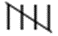10 164 ||| 3 165 ||| 3 168 | 1 Total 25

Therefore median = ((n+1)/2)th term

Median = value of 13th term

Median = 163 cm

Here, clearly, 163 cm occurs the most number of times. Therefore, the mode of the given data is 163 cm.

Mode = 3 Median – 2 Mean

163 = 3 x 163 – 2 Mean

2 Mean = 326

Mean = 163 cm.

7. The scores in mathematics test (out of 25) of 15 students are as follows:

19, 25, 23, 20, 9, 20, 15, 10, 5, 16, 25, 20, 24, 12, 20

Find the mode and median of this data. Are they same?

Solution:

Arranging the data in ascending order such that same values are put together, we get:

5, 9, 10, 12, 15, 16, 19, 20, 20, 20, 20, 23, 24, 25, 25

Here, n = 15

Therefore median = ((n+1)/2)th term

Median = value of 8th term

Median = 20

Here, clearly, 20 occurs most number of times, i.e., 4 times. Therefore, the mode of the given data is 20.

Yes, the median and mode of the given data are the same.

8. Calculate the mean and median for the following data:

 Marks 10 11 12 13 14 16 19 20 Number of students 3 5 4 5 2 3 2 1

Using empirical formula, find its mode.

Solution:

Calculation of mean

Mean = Σ fi xi/ Σ fi

= 332/25

= 13.28

Here, n = 25, which is an odd number. Therefore,

Therefore median = ((n+1)/2)th term

Median = value of 13th term

Median = 13

Now, by using empirical formula we have,

Mode = 3Median – 2 Mean

Mode = 3 (13) – 2 (13.28)

Mode = 39 – 26.56

Mode = 12.44.

9. The following table shows the weights of 12 persons.

 Weight (in kg) 48 50 52 54 58 Number of persons 4 3 2 2 1

Find the median and mean weights. Using empirical relation, calculate its mode.

Solution:

 xi fi xi fi 48 4 192 50 3 150 52 2 104 54 2 108 58 1 58 Total Σ fi = 12 Σ fi xi = 612

Calculation of mean

Mean = Σ fi xi/ Σ fi

= 612/12

= 51 kg

Here n = 12

Therefore median = (n/2)th term + ((n + 1)/2)th term

Median = (value of 6th term + value of 7th term)/2

= (50 + 50)/2

= 50

Now by empirical formula we have,

Now,

Mode = 3 Median – 2 Mean

Mode = 3 x 50 – 2 x 51

Mode = 150 – 102

Mode = 48 kg.

Thus, Mean = 51 kg, Median = 50 kg and Mode = 48 kg.